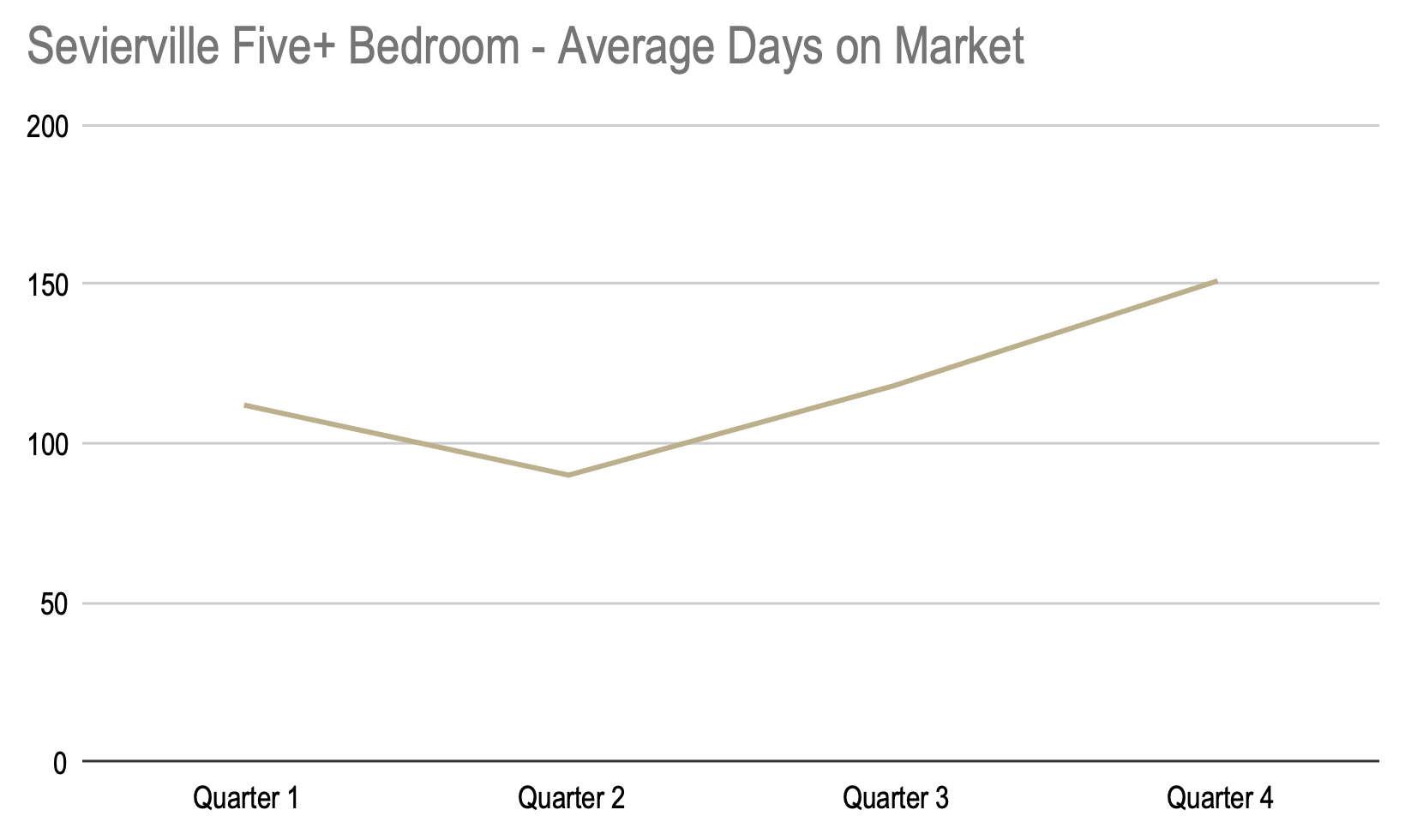# 2021 Real Estate Market Statistics – Gatlinburg, Pigeon Forge, Sevierville

## 2021 Real Estate Market Statistics – Gatlinburg, Pigeon Forge, Sevierville

Below you will find statistics for Gatlinburg, Pigeon Forge, and Sevierville separated by bedroom!

## 2021 Real Estate Market Statistics – Gatlinburg

#### One Bedroom

We began the year with an average sales price of \$311,558 and ended the year at an average price of \$408,171.00. For a total increase of \$96,613.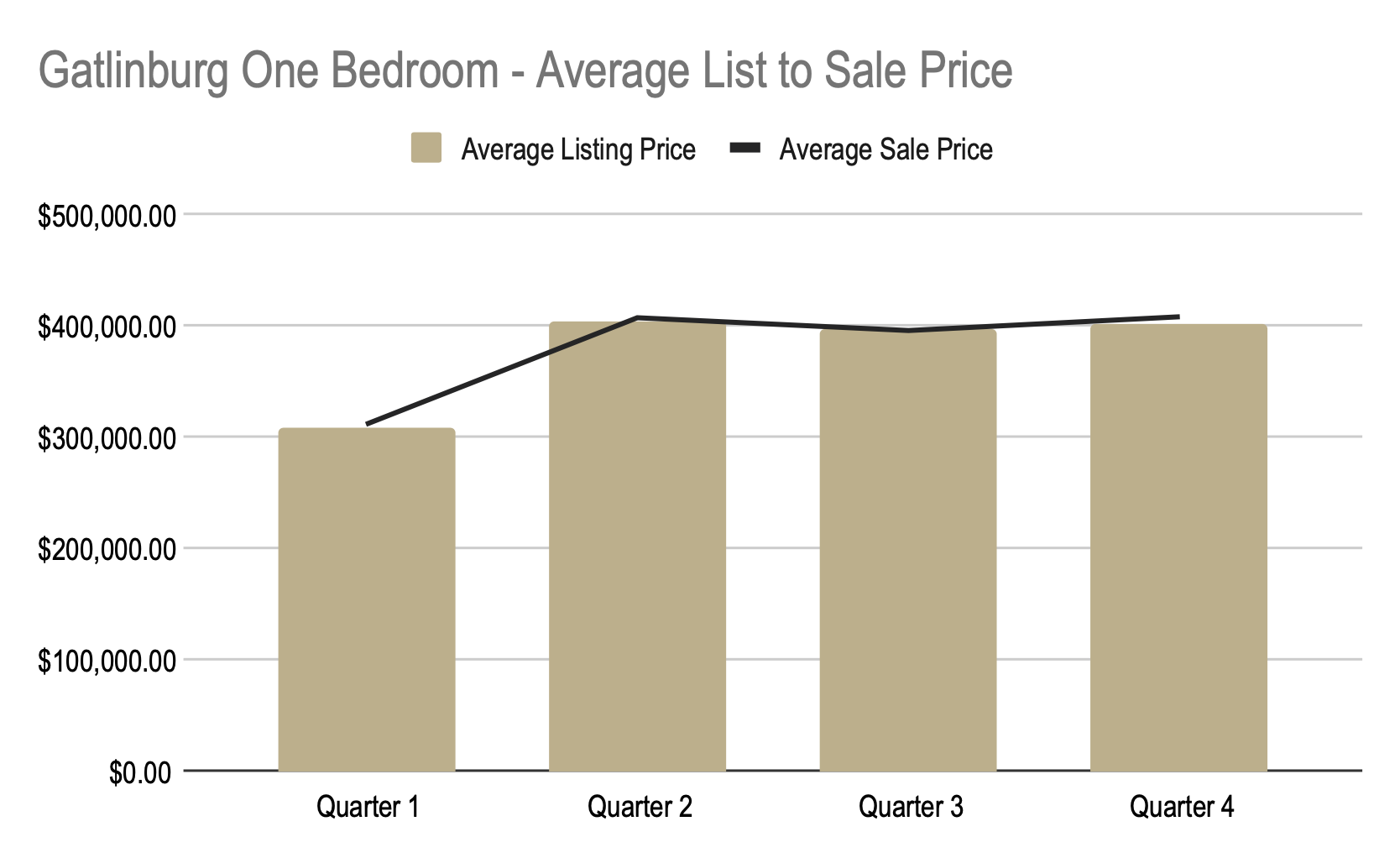We began the year at \$317.80 a square foot and ended the year at \$452.17 a square foot. For a total increase of \$134.37 per square foot.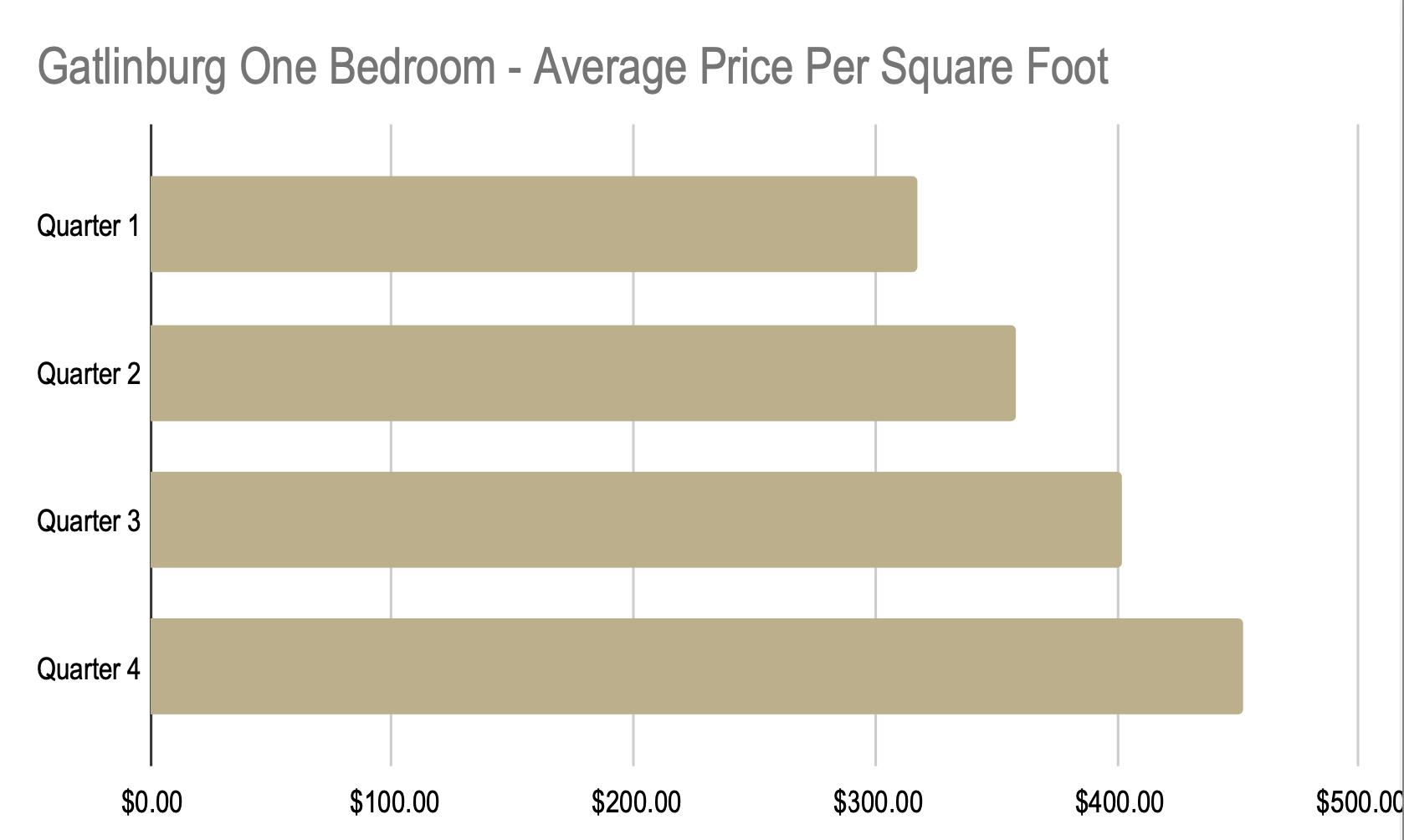We began the year at 46 days on market and ended the year at 70 days on market. For a total increase of 24 days.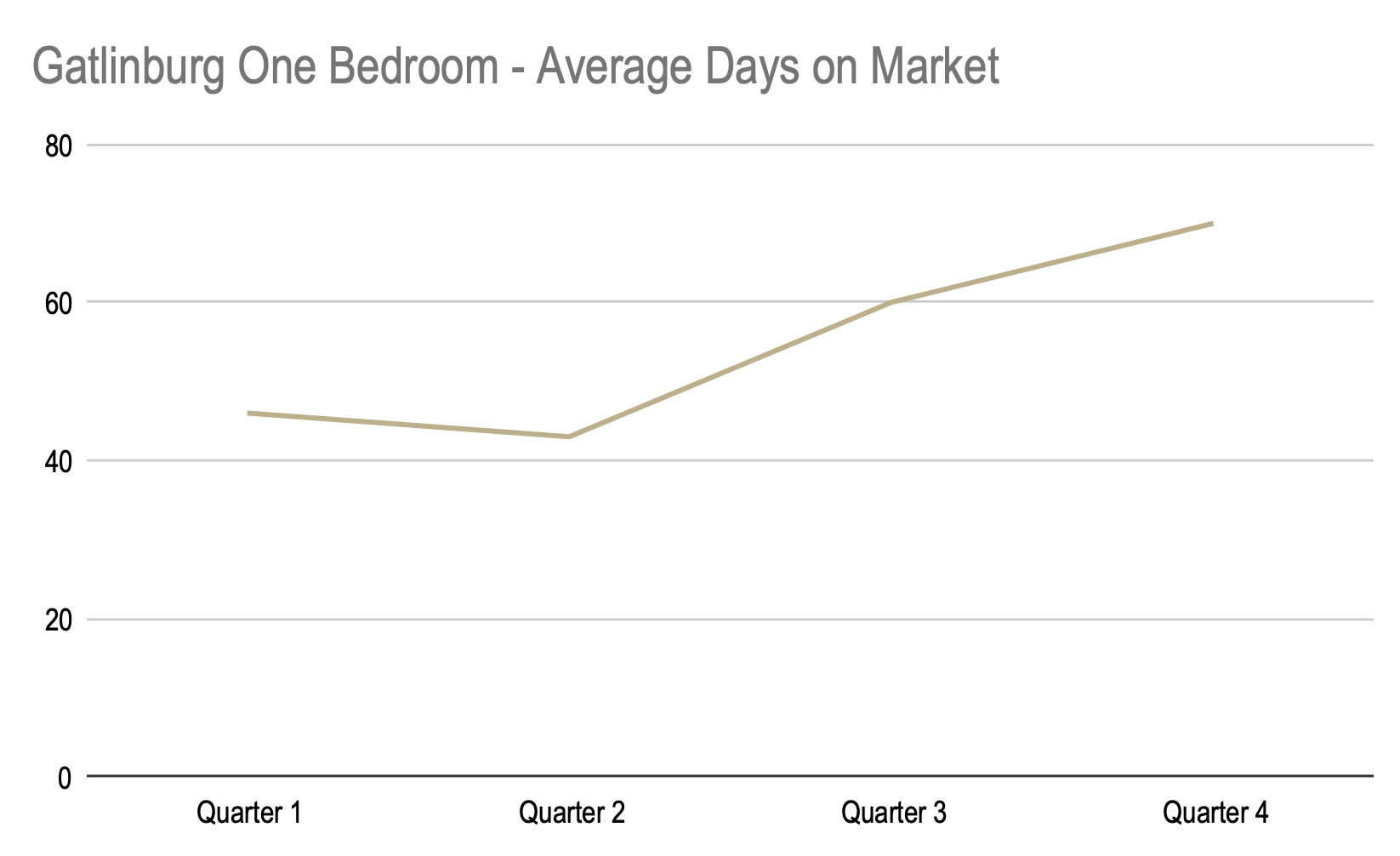### Two Bedroom

We began the year with an average sales price of \$405,956.00 and ended the year at an average price of \$491,147. For a total increase of \$85,191.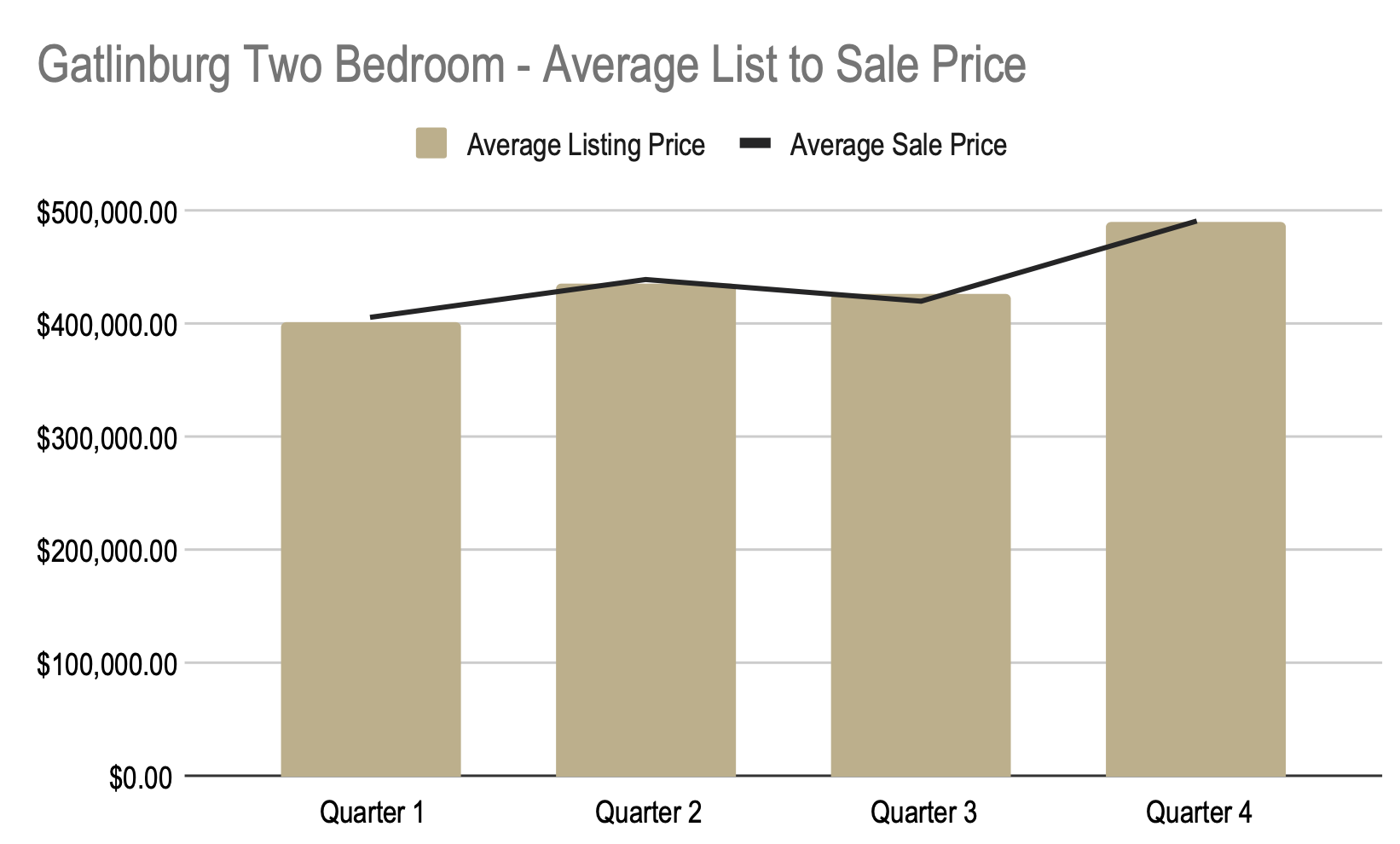We began the year at \$278.43 a square foot and ended the year at \$351.29 a square foot. For a total increase of \$72.86 per square foot.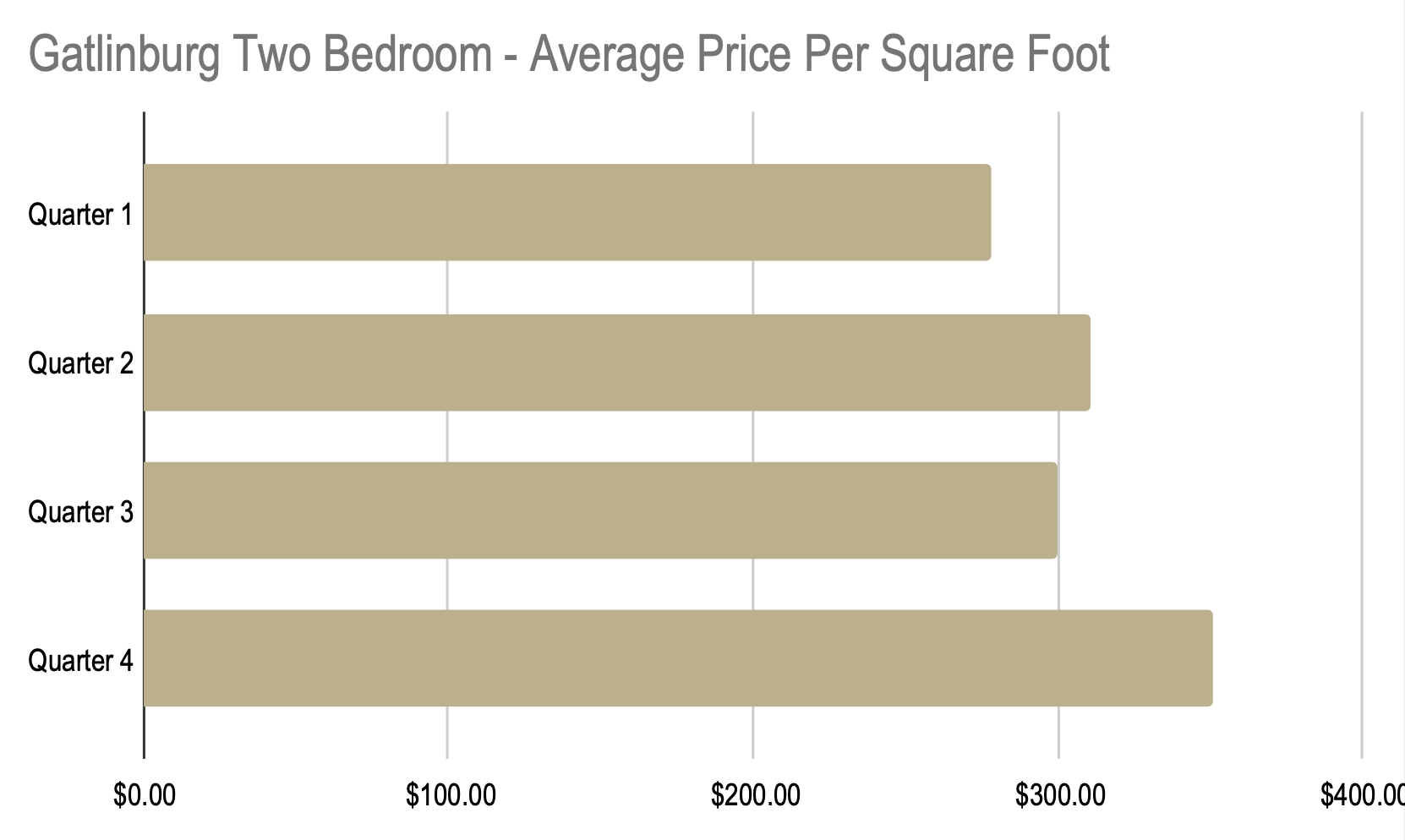We began the year at 31 days on market and ended the year at 31 days on market. For a total increase of 53 days.### Three Bedroom

We began the year with an average sales price of \$516,184 and ended the year at an average price of \$679,552. For a total increase of \$163,368.We began the year at \$260 a square foot and ended the year at \$310 a square foot. For a total increase of \$51 per square foot.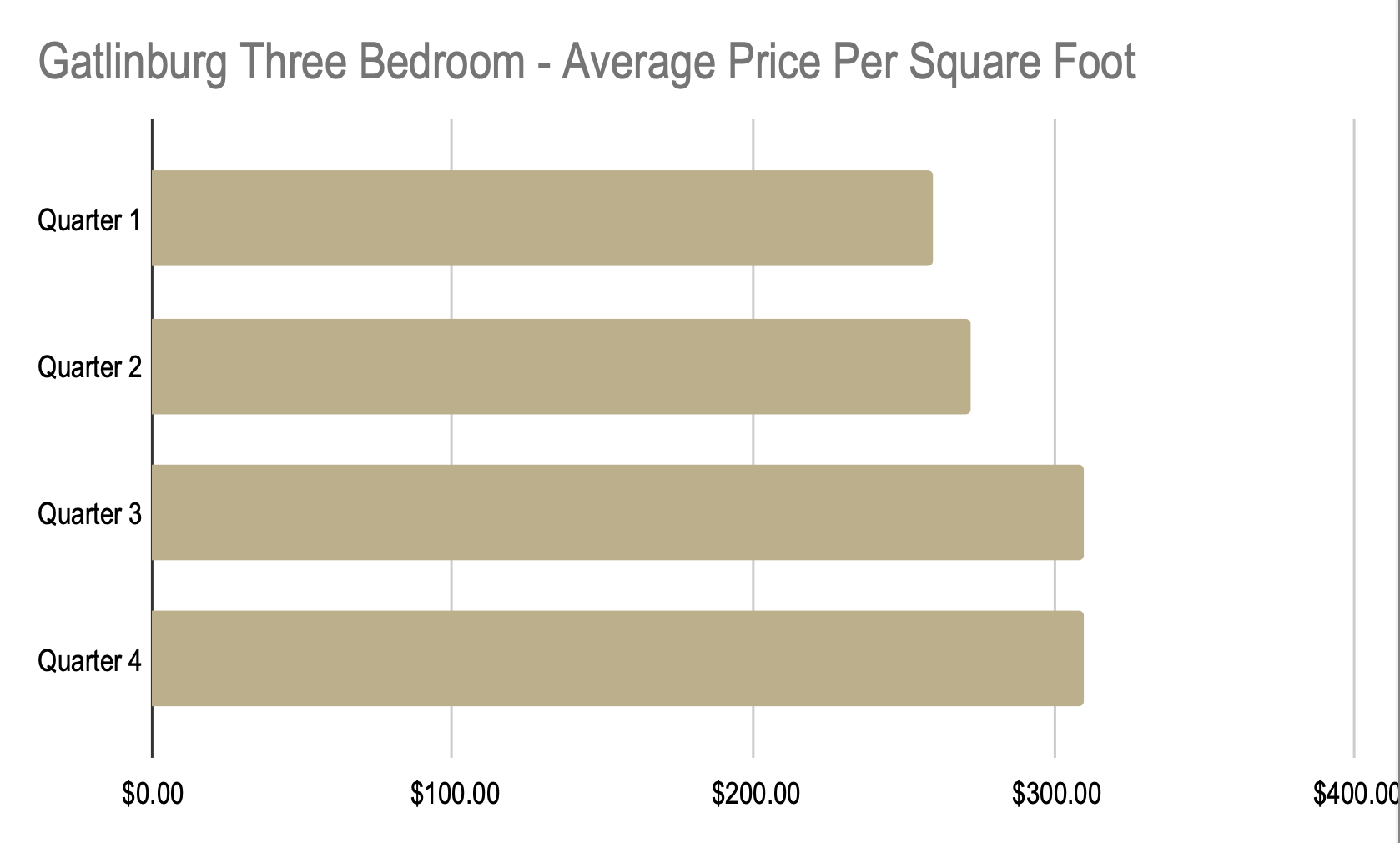We began the year at 80 days on market and ended the year at 103 days on market. For a total increase of 23 days.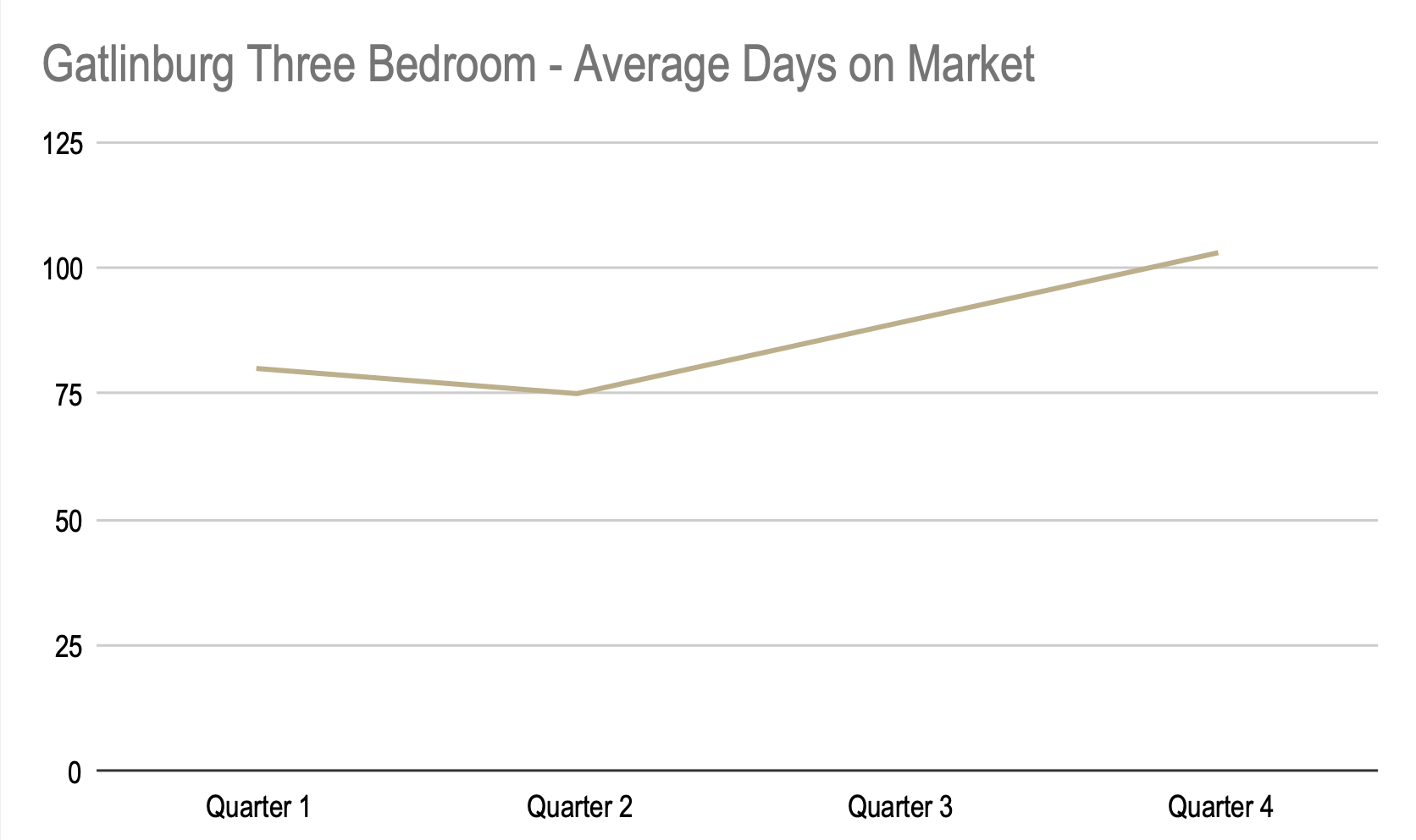### Four Bedroom

We began the year with an average sales price of \$849,567 and ended the year at an average price of \$762,303. For a total increase of \$87,264.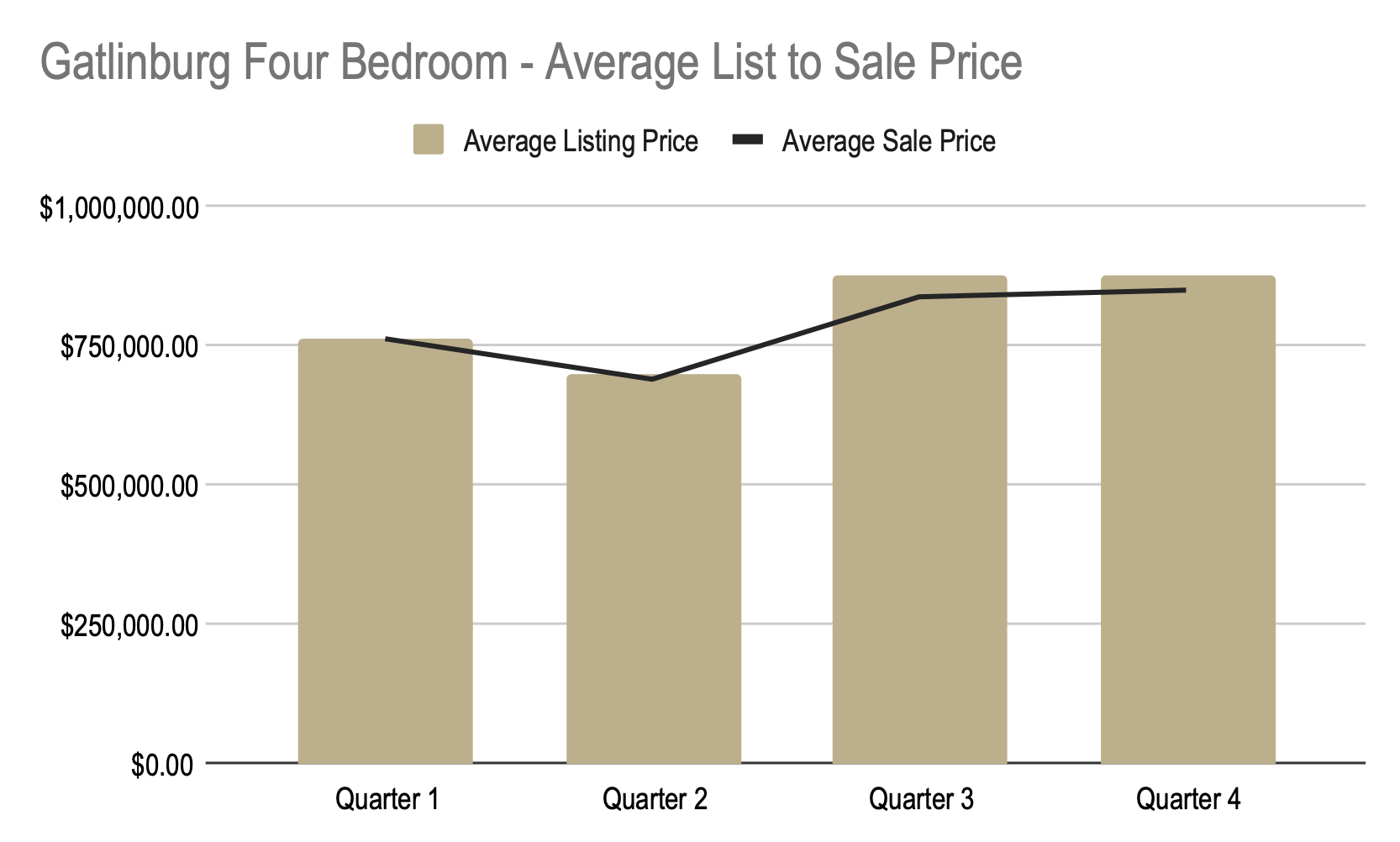We began the year at \$284 a square foot and ended the year at \$289 a square foot. For a total increase of \$5 per square foot.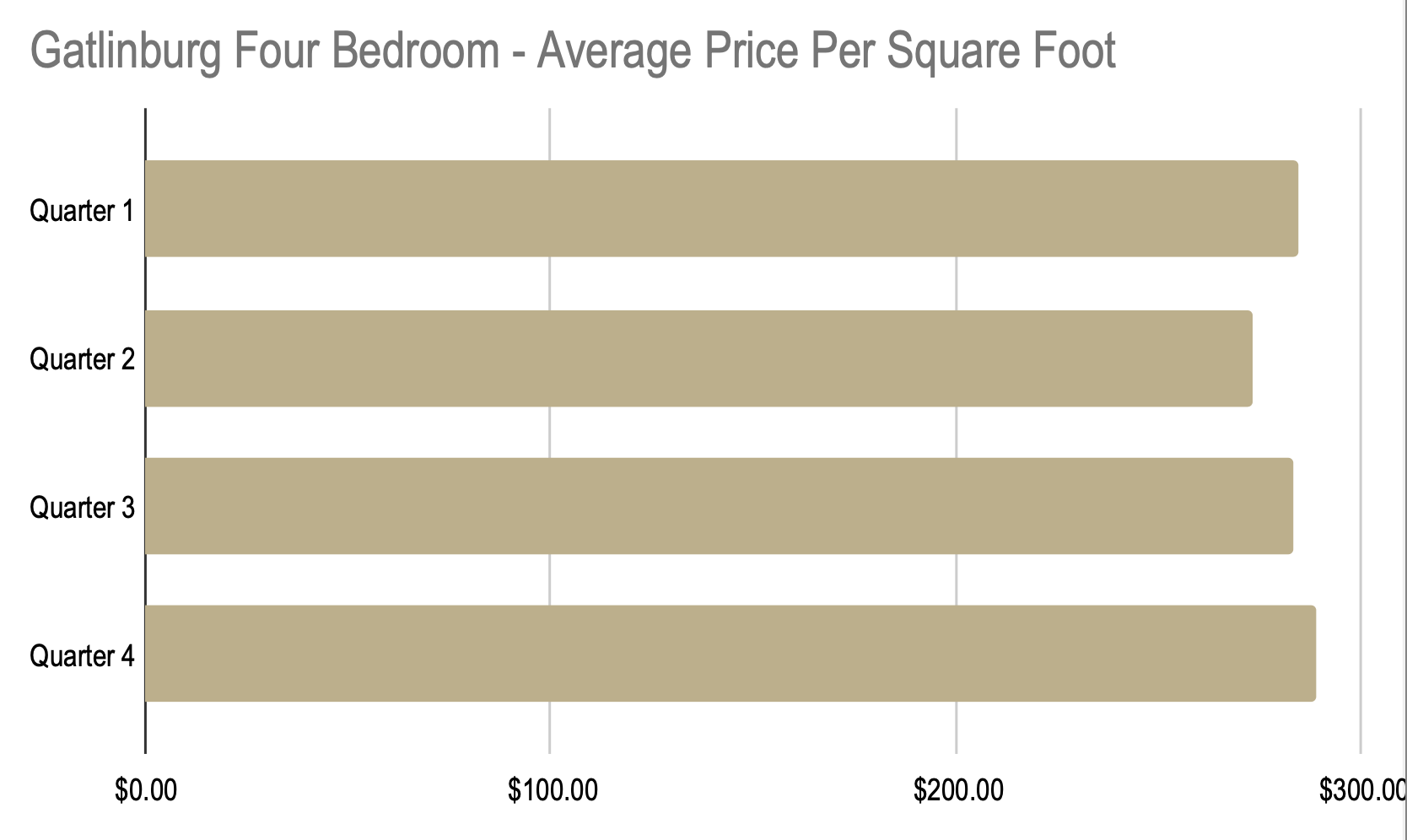We began the year at 74 days on market and ended the year at 154 days on market. For a total increase of 80 days.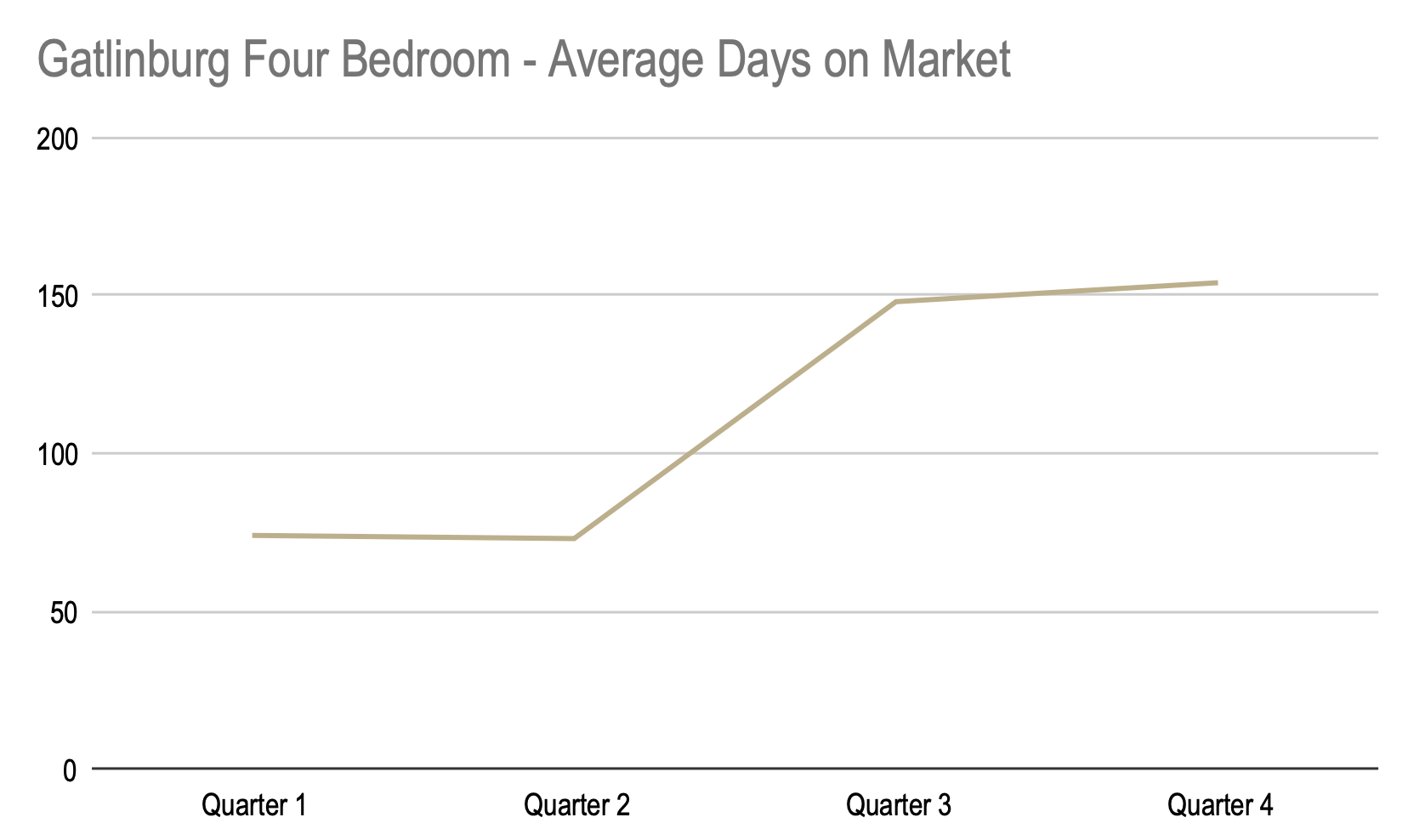### Five+ Bedroom

We began the year with an average sales price of  \$932,164 and ended the year at an average price of \$1,339,262. For a total increase of \$407,098.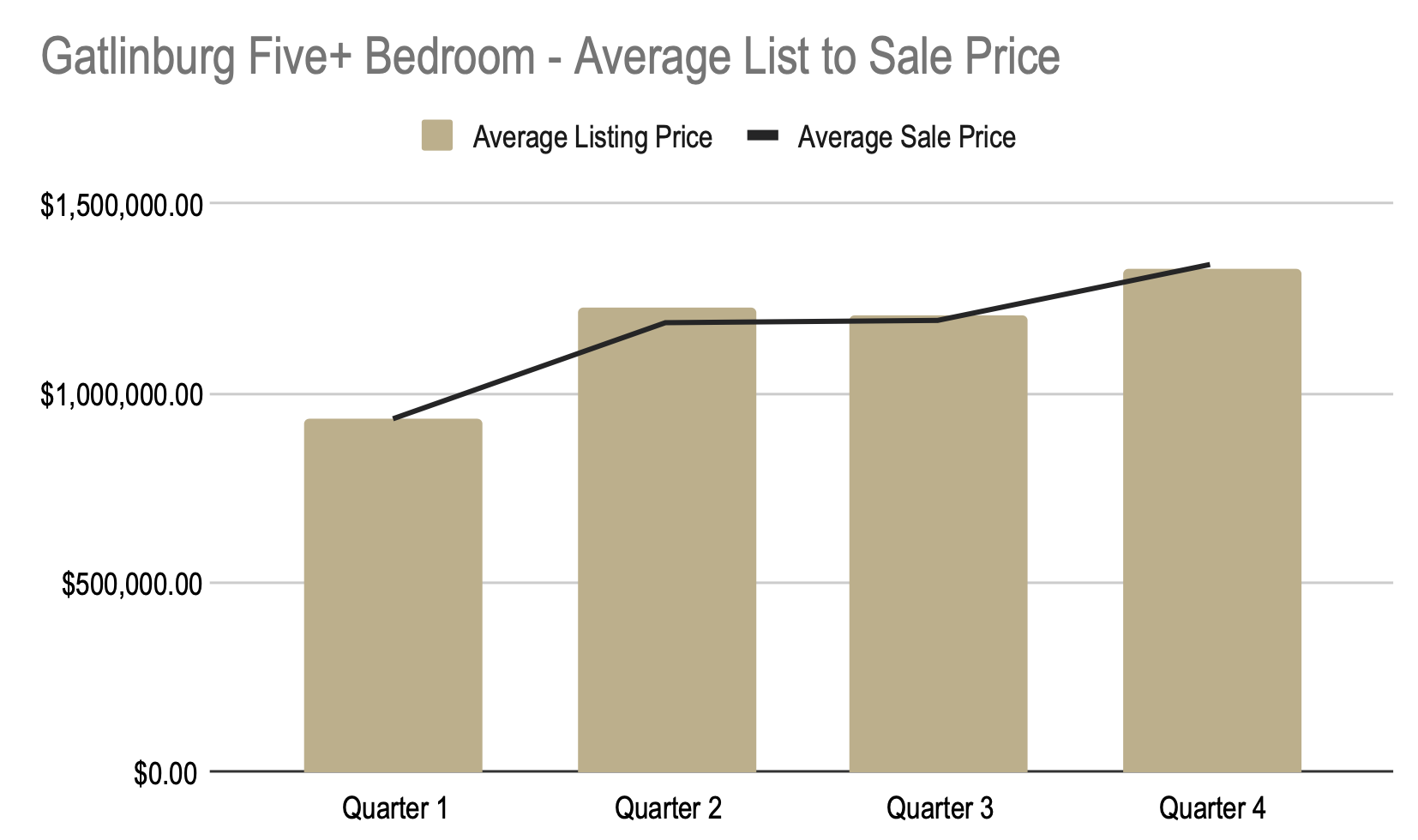We began the year at \$237 a square foot and ended the year at \$334 a square foot. For a total increase of \$97 per square foot.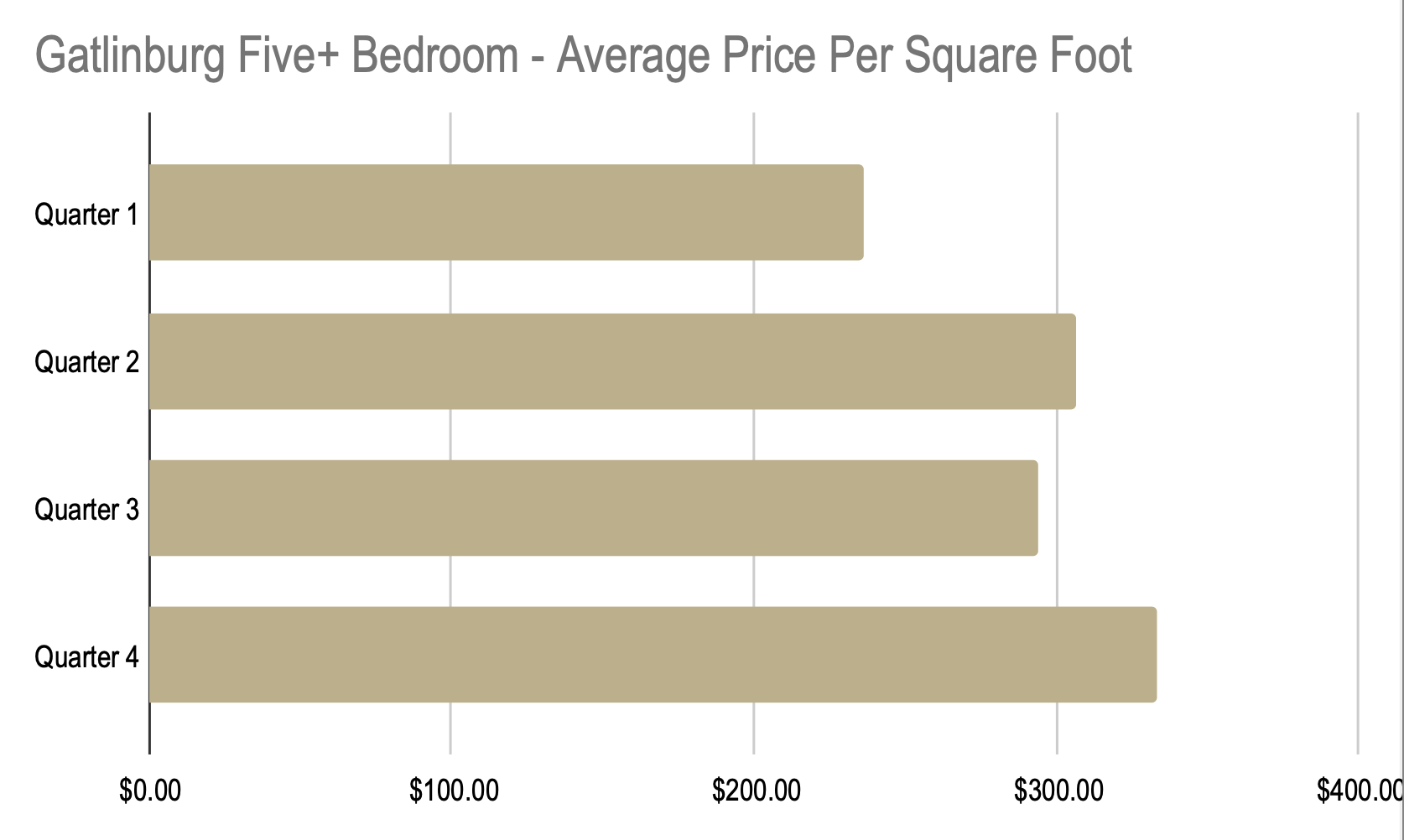We began the year at 41 days on market and ended the year at 113 days on market. For a total increase of 72 days.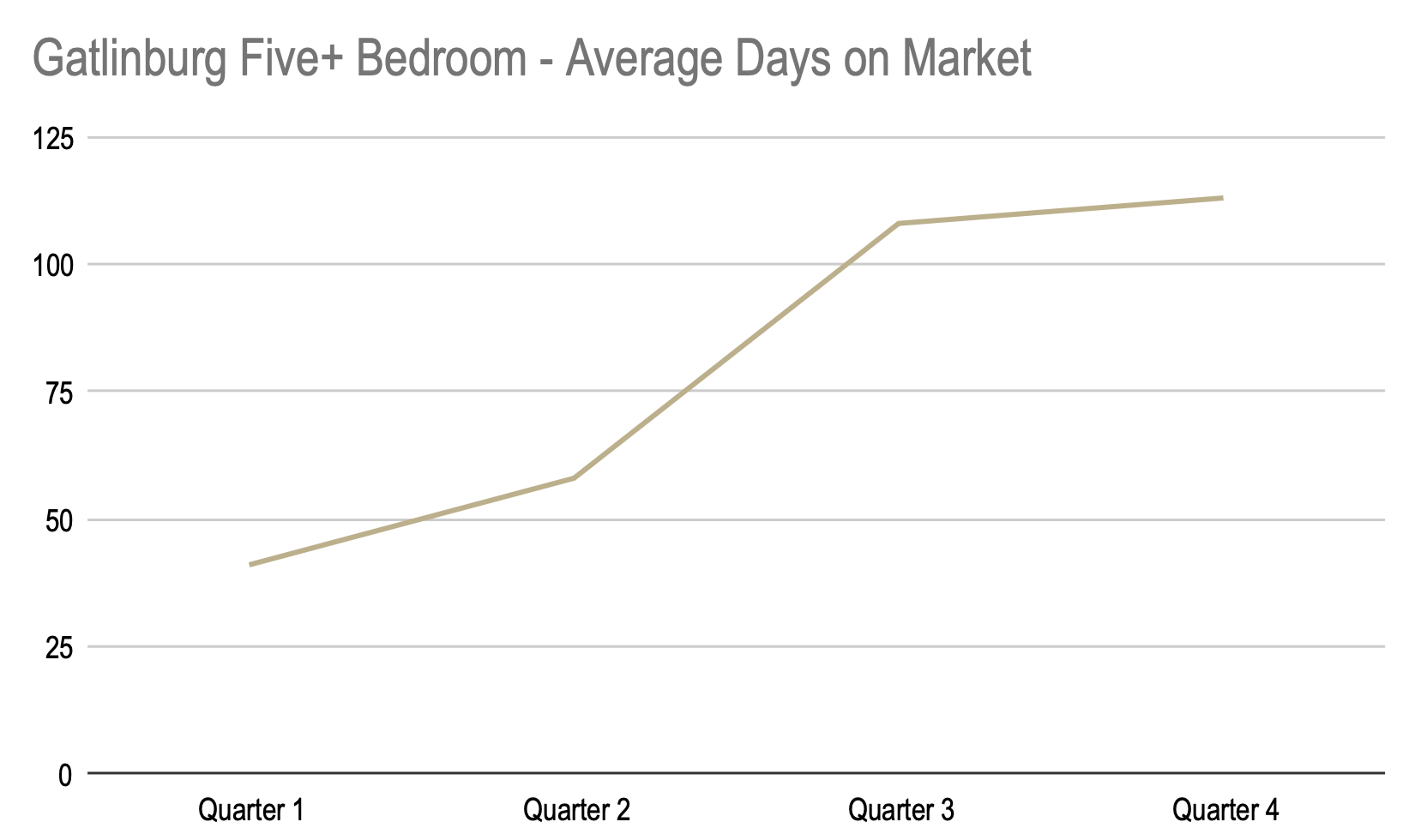## 2021 Real Estate Market Statistics – Pigeon Forge

#### One Bedroom

We began the year with an average sales price of \$350,973 and ended the year at an average price of \$439,286. For a total increase of \$88,313.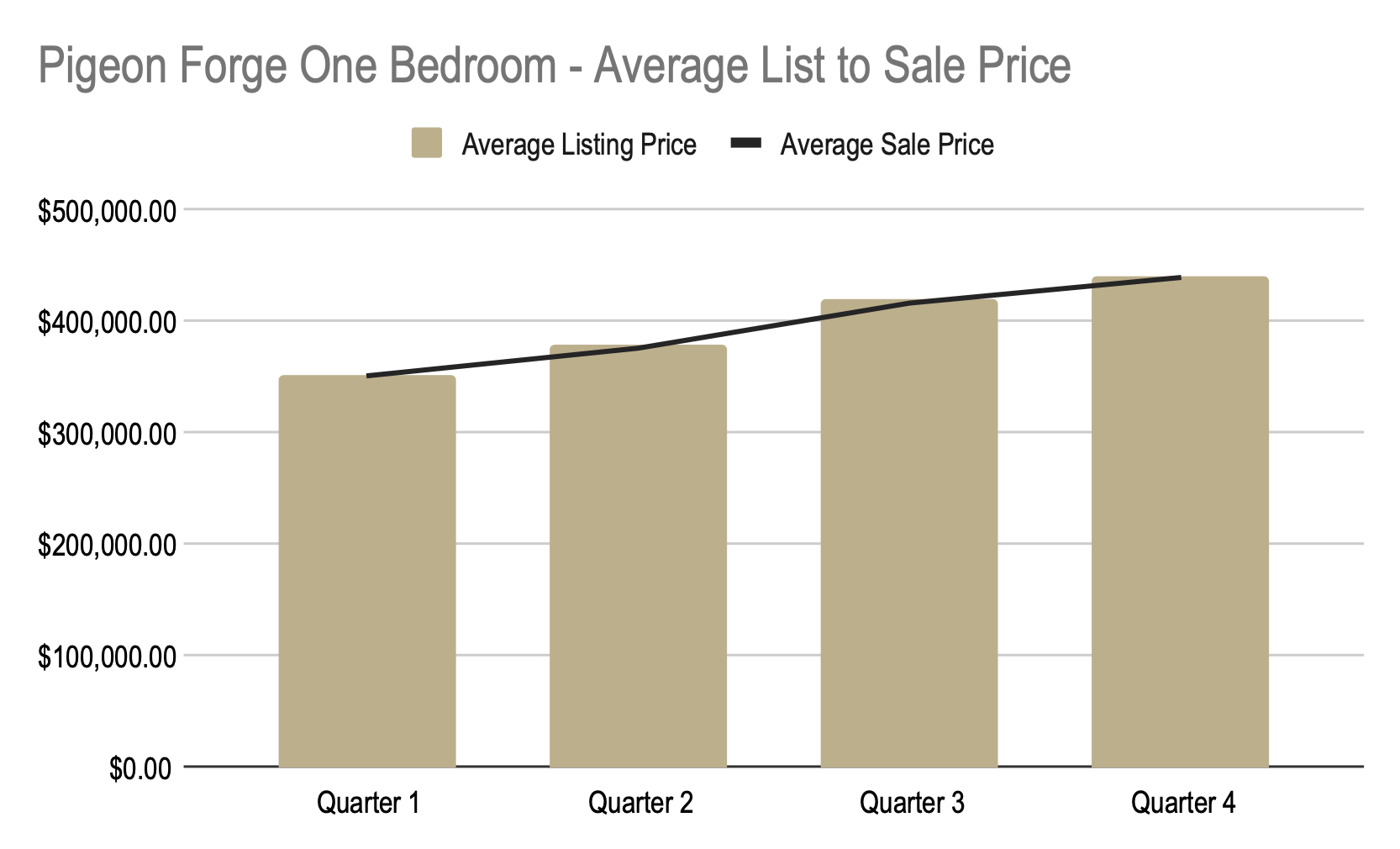We began the year at \$346 a square foot and ended the year at \$431 a square foot. For a total increase of \$84 per square foot.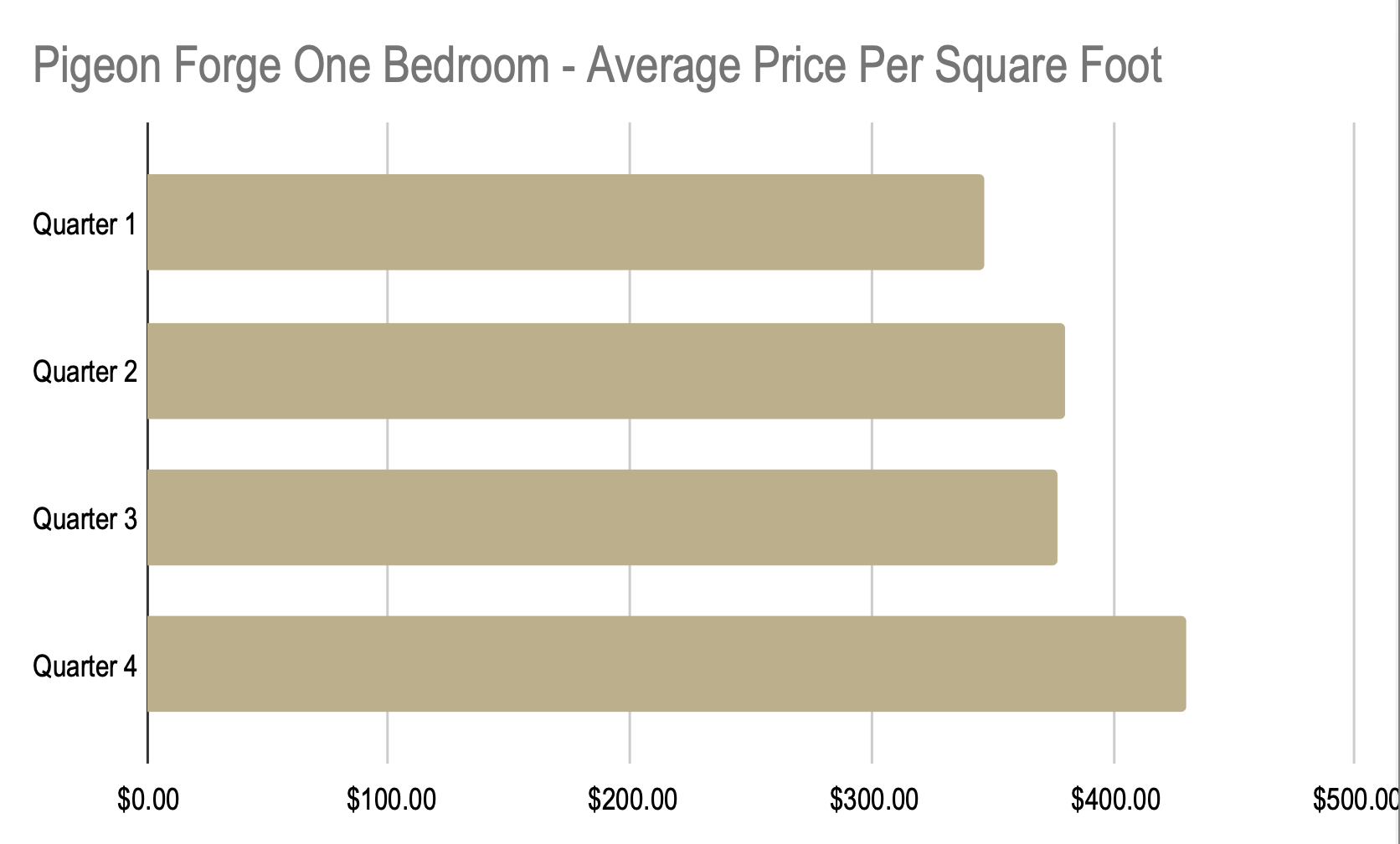We began the year at 42days on market and ended the year at 64 days on market. For a total increase of 22 days.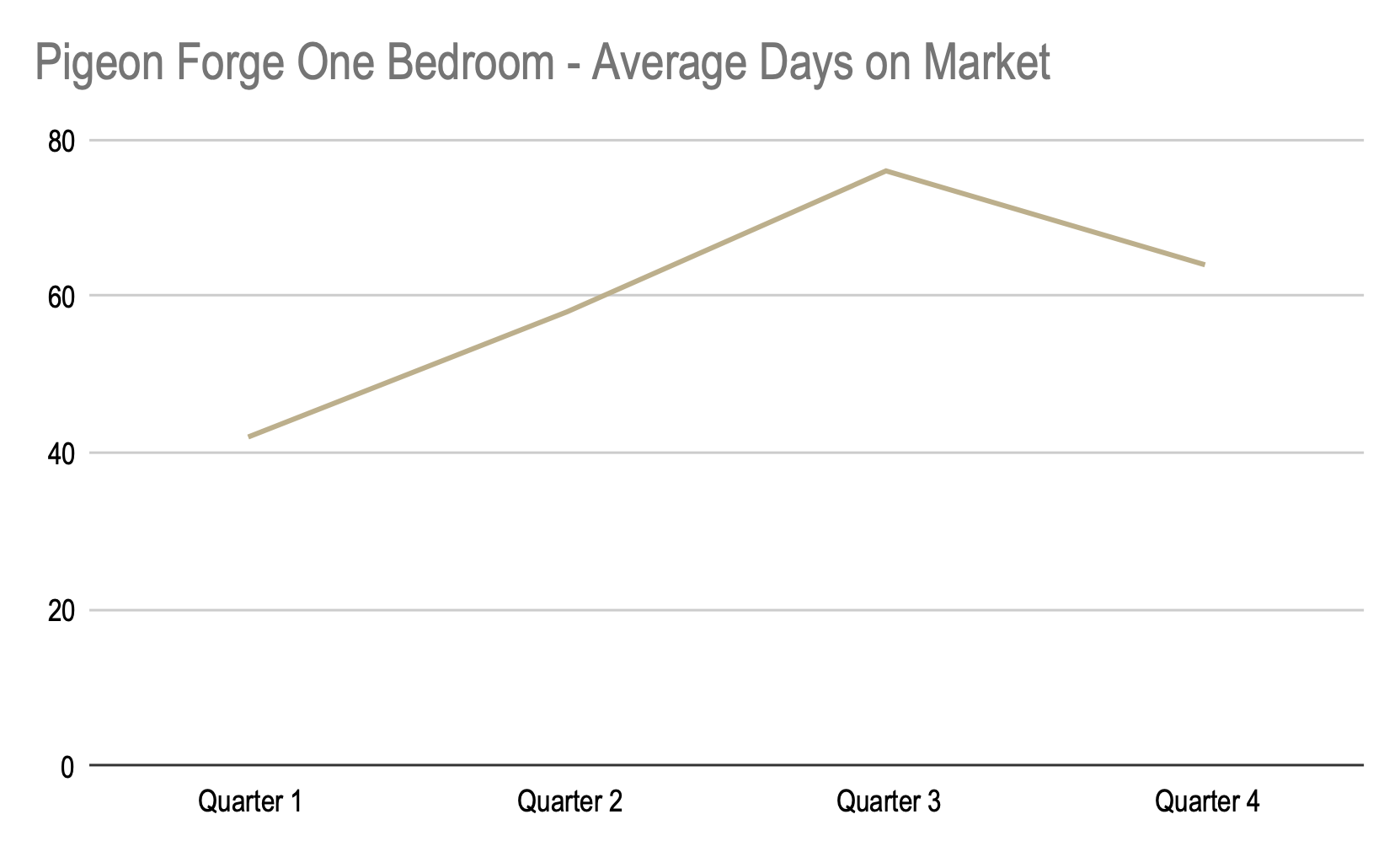### Two Bedroom

We began the year with an average sales price of \$393,243 and ended the year at an average price of \$527,686 For a total increase of \$134,443.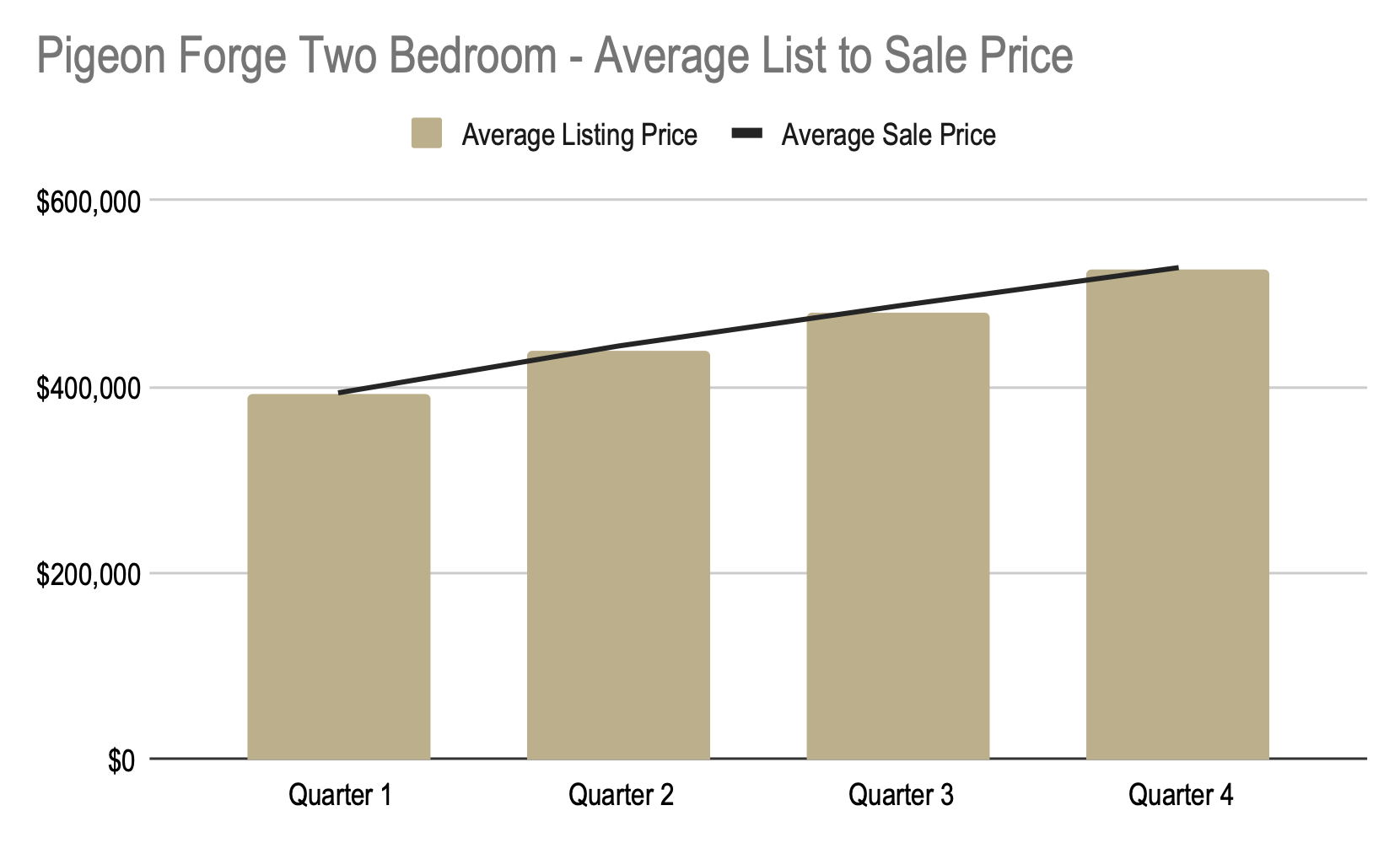We began the year at \$278 a square foot and ended the year at \$346 a square foot. For a total increase of \$68 per square foot.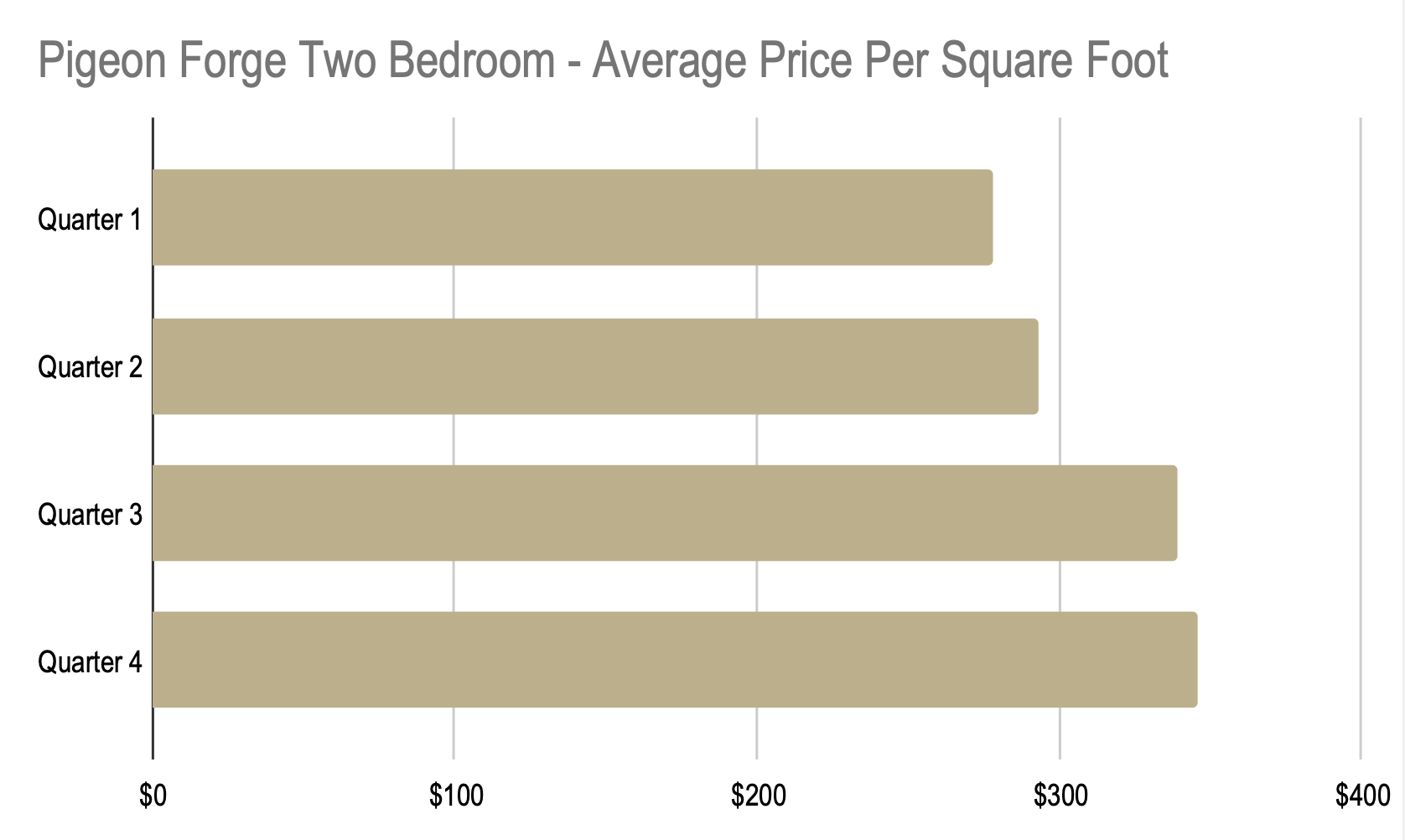We began the year at 46 days on market and ended the year at 86 days on market. For a total increase of 40 days.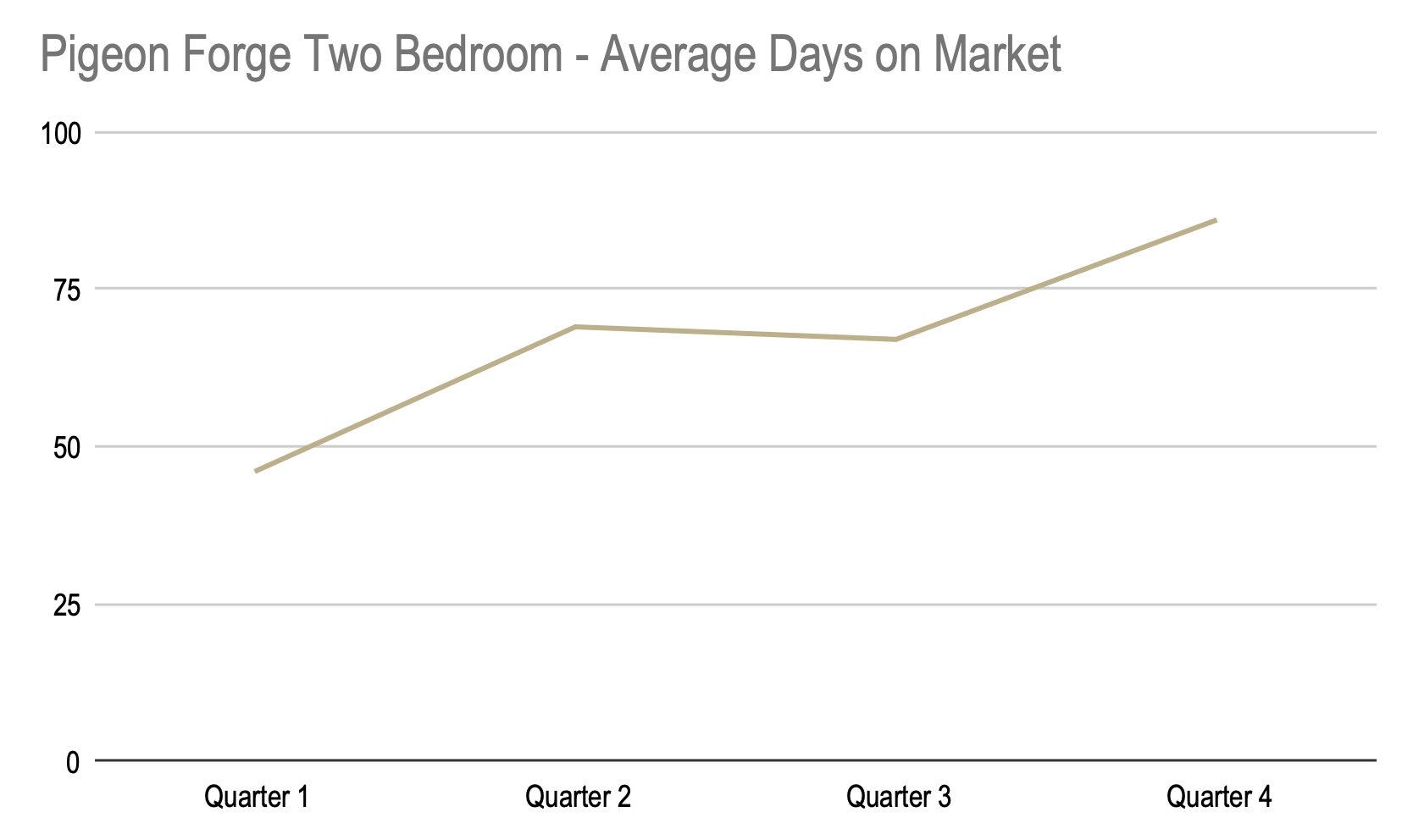### Three Bedroom

We began the year with an average sales price of \$510,142 and ended the year at an average price of \$734,256. For a total increase of \$224,114.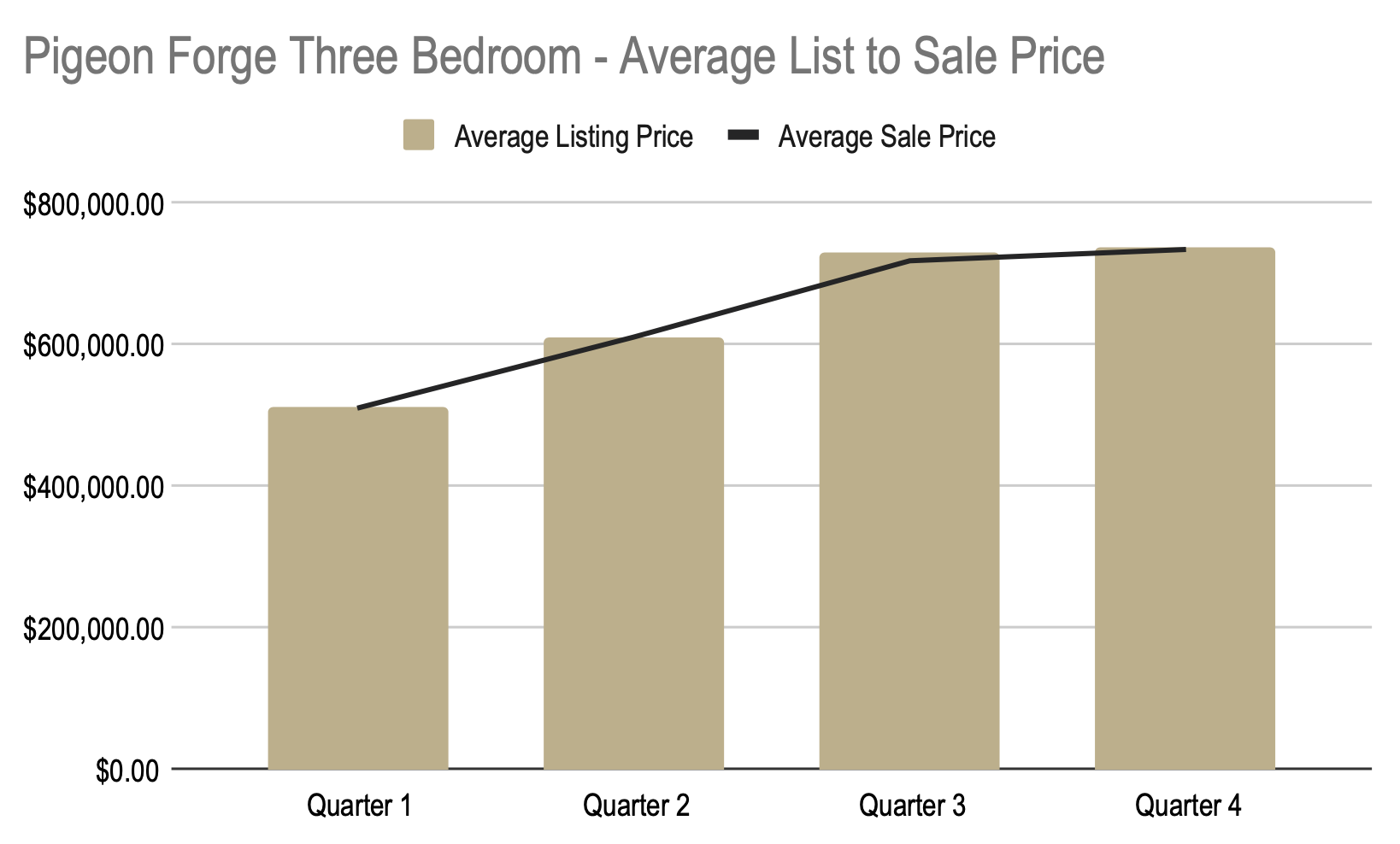We began the year at \$239 a square foot and ended the year at \$313 a square foot. For a total increase of \$74 per square foot.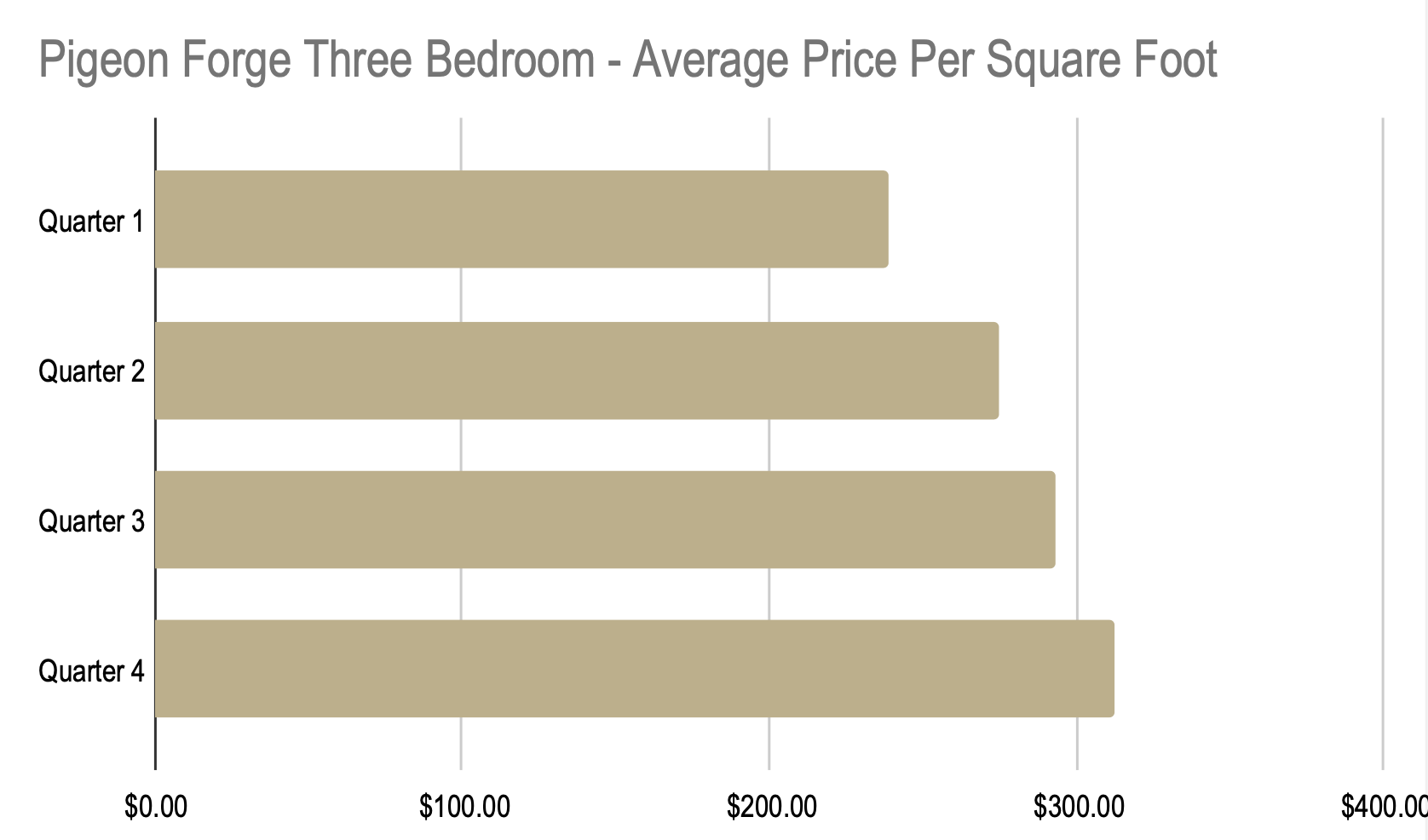We began the year at 90 days on market and ended the year at 104 days on market. For a total increase of 14 days.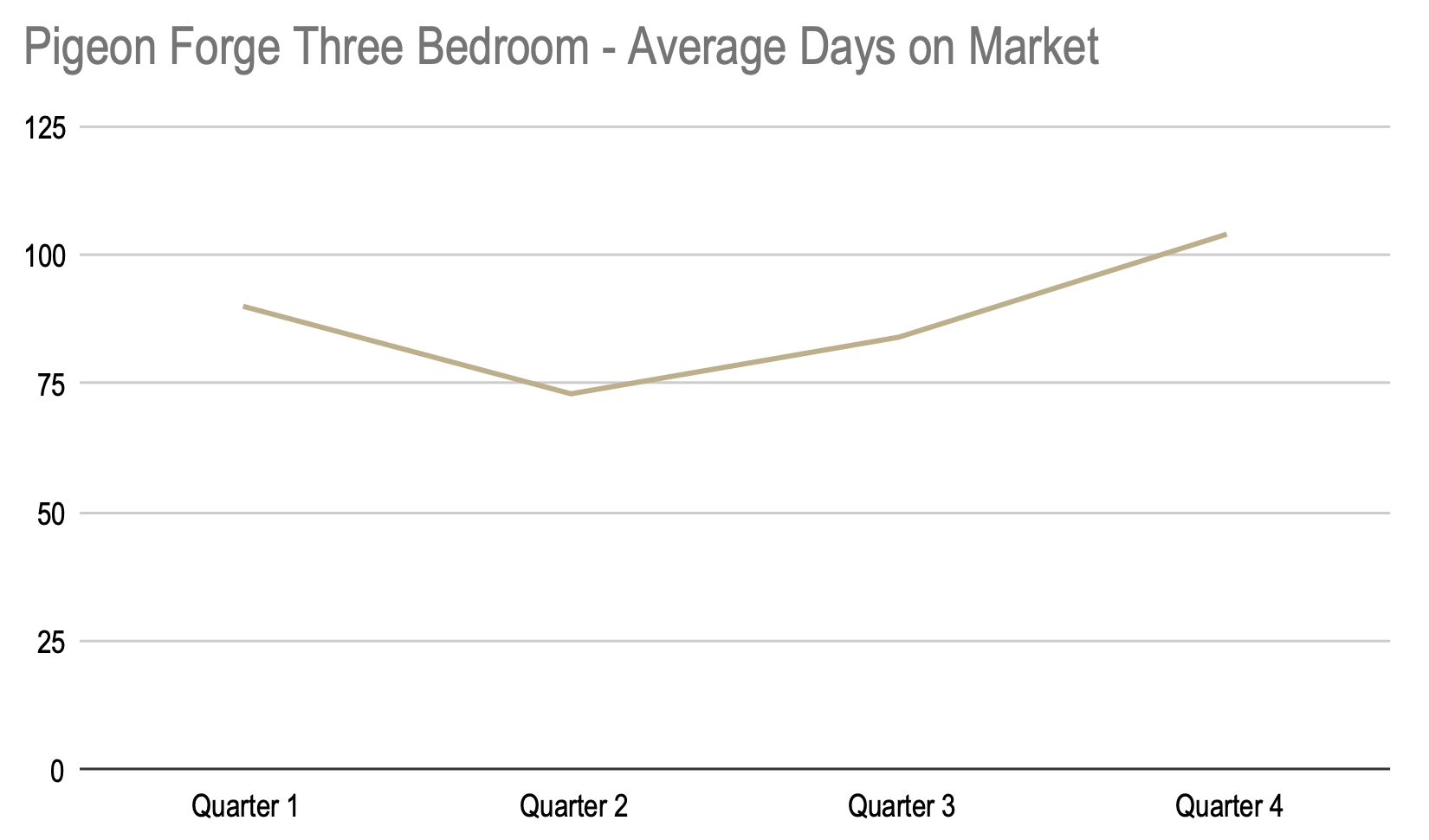### Four Bedroom

We began the year with an average sales price of \$677,152 and ended the year at an average price of \$935,960. For a total increase of \$258,808.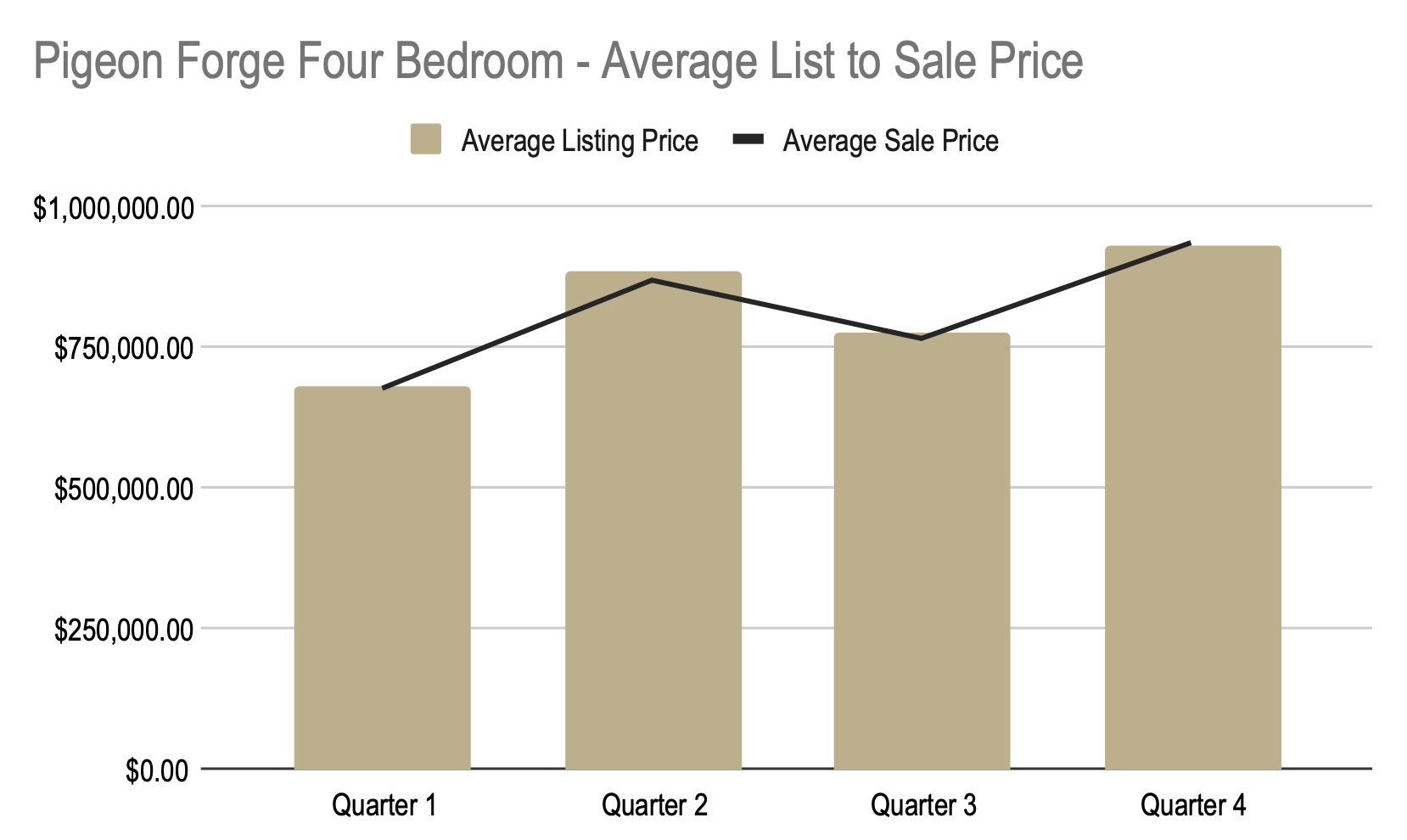We began the year at \$244 a square foot and ended the year at \$331 a square foot. For a total increase of \$87 per square foot.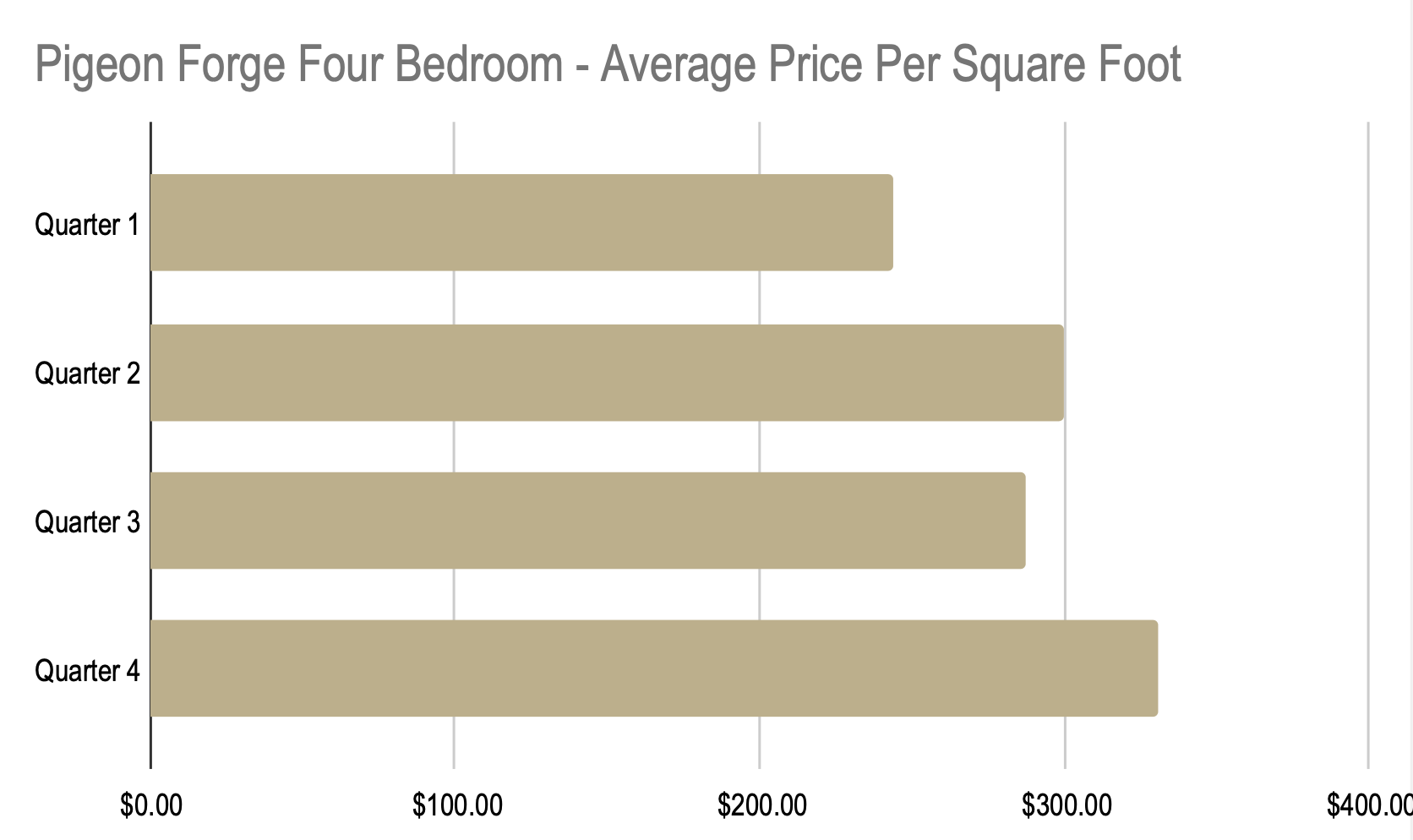We began the year at 89 days on market and ended the year at 198 days on market. For a total increase of 109 days.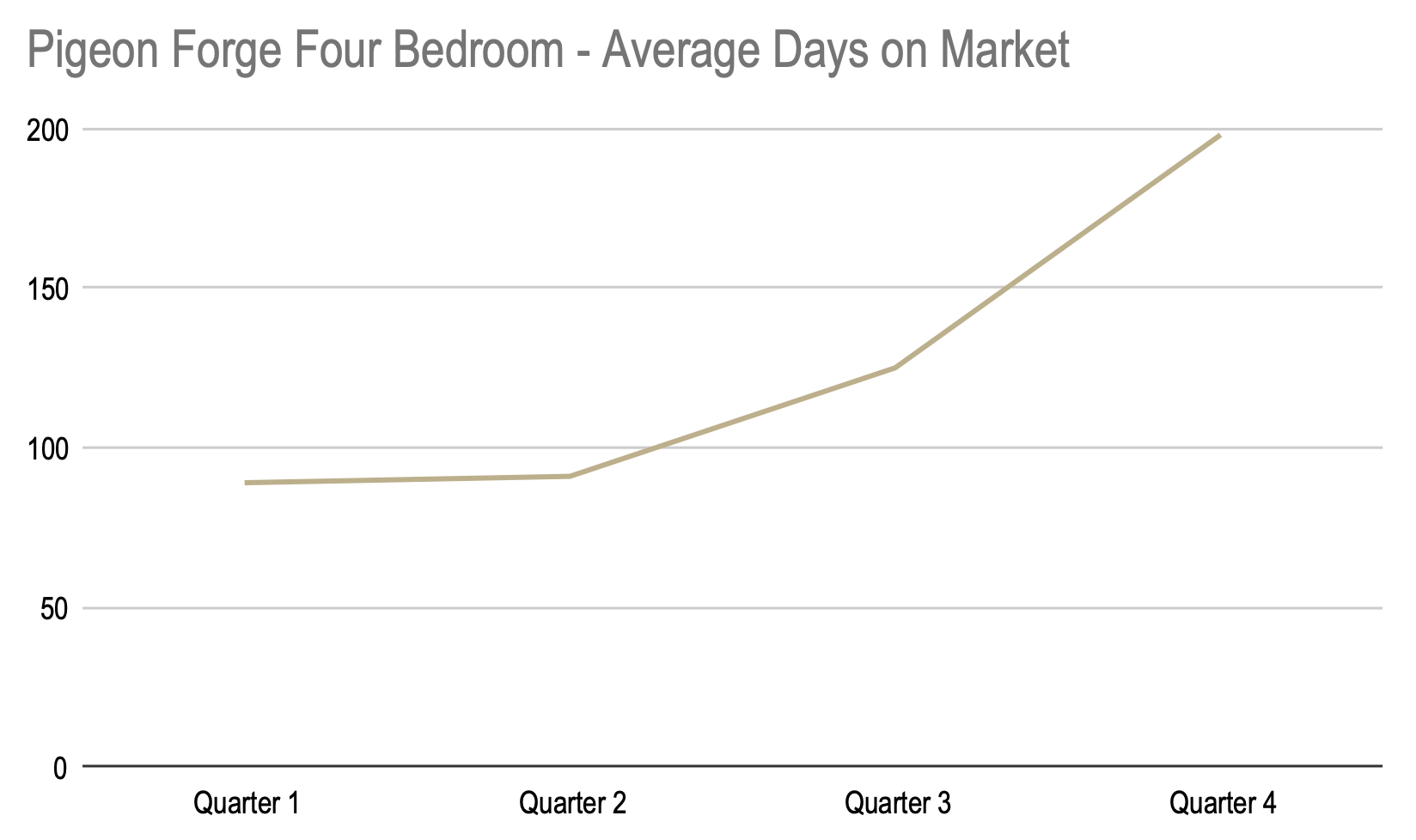### Five+ Bedroom

We began the year with an average sales price of \$770,025 and ended the year at an average price of \$1,303,848. For a total increase of \$533,823.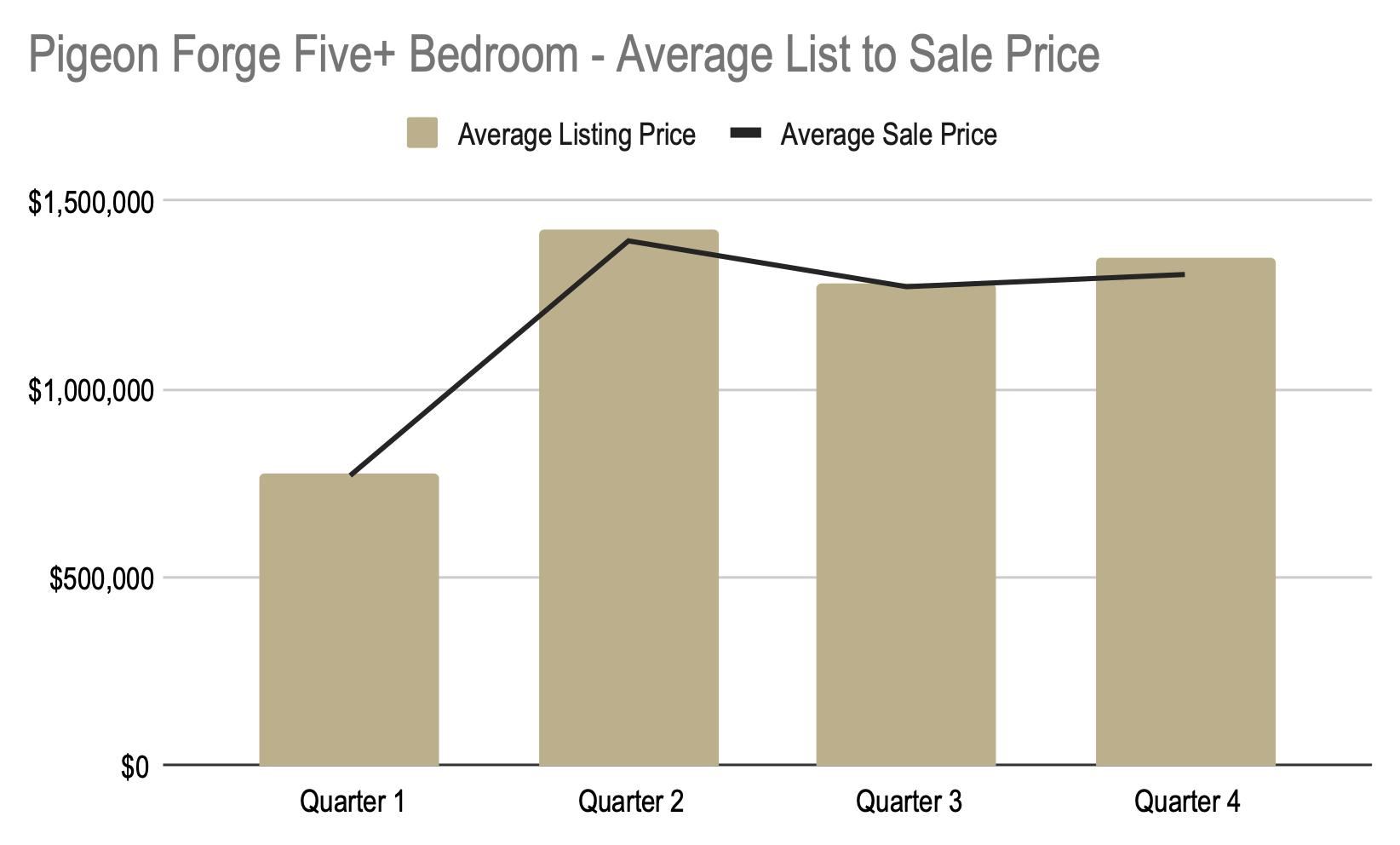We began the year at \$211 a square foot and ended the year at \$321 a square foot. For a total increase of \$110 per square foot.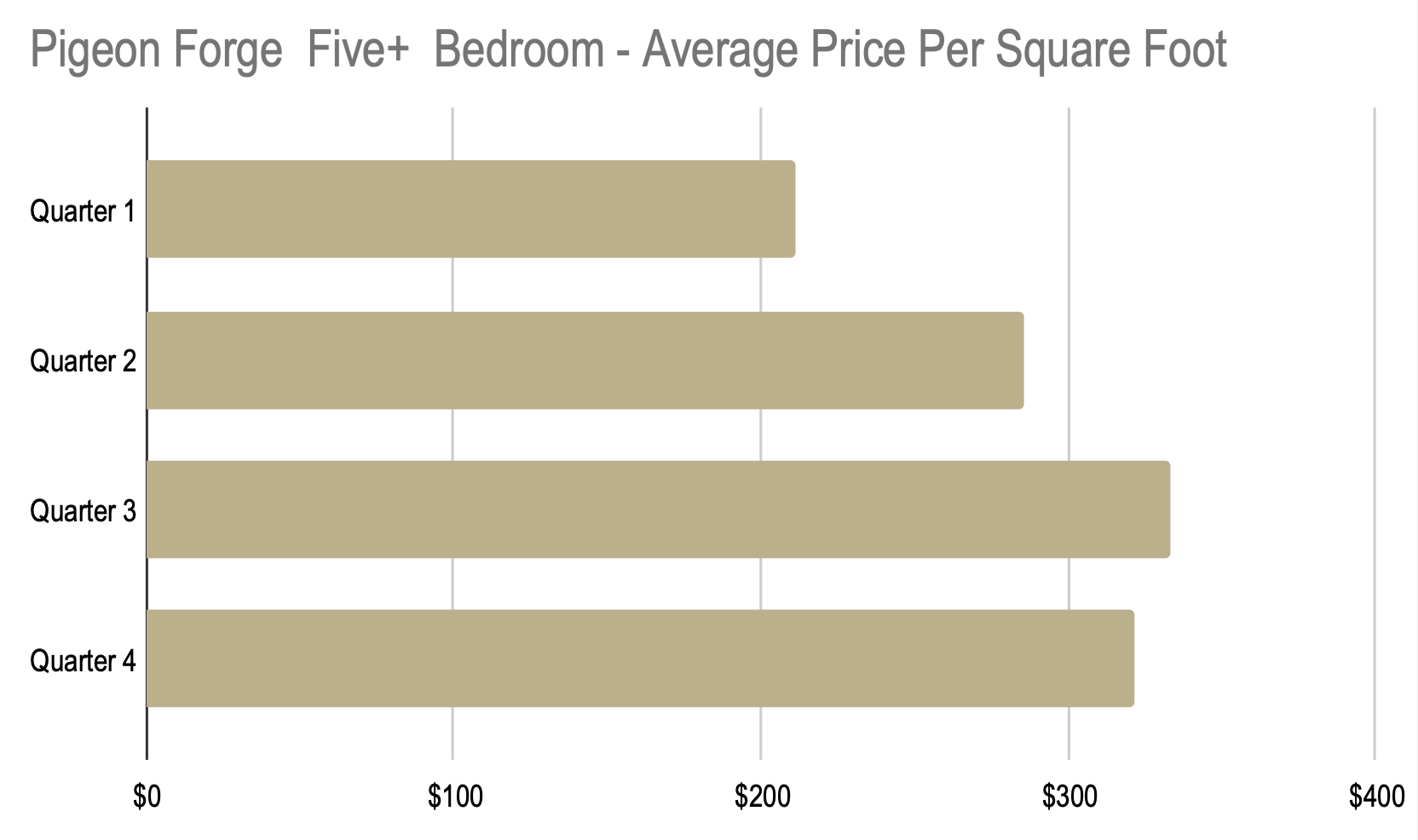We began the year at 129 days on market and ended the year at 139 days on market. For a total increase of 10 days.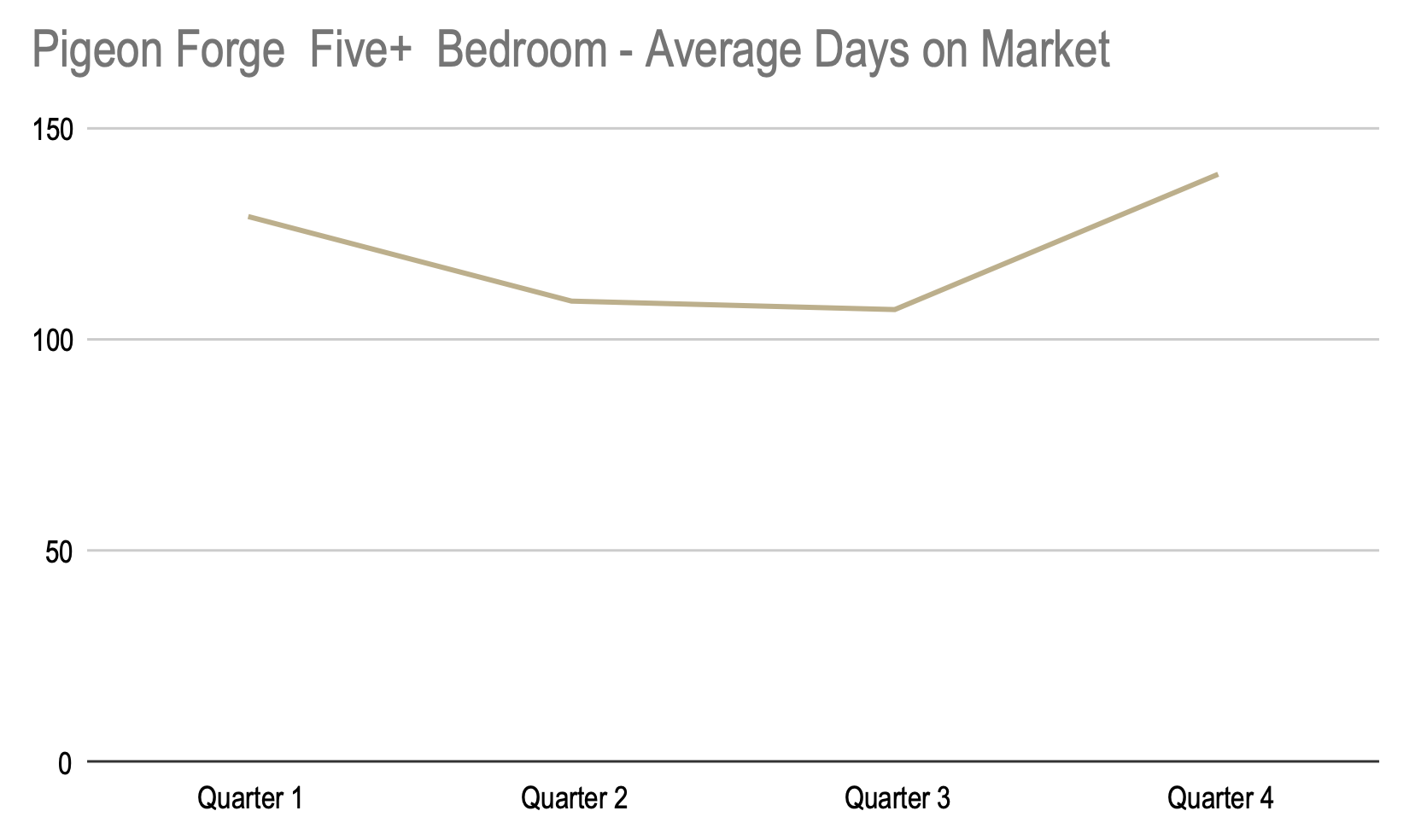## 2021 Real Estate Market Statistics – Sevierville

#### One Bedroom

We began the year with an average sales price of \$303,200 and ended the year at an average price of \$375,837. For a total increase of \$72,637.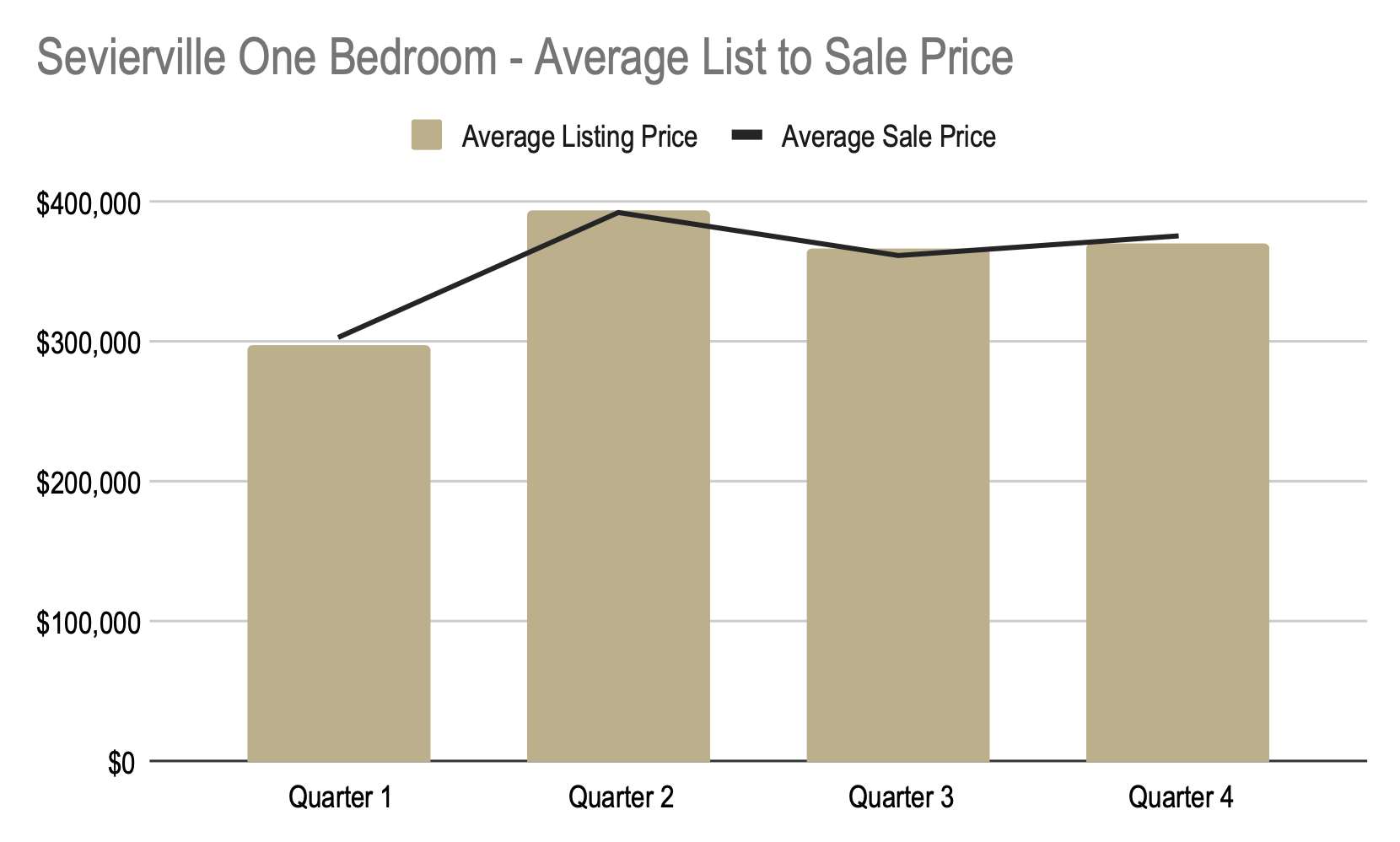We began the year at \$293 a square foot and ended the year at \$400 a square foot. For a total increase of \$108per square foot.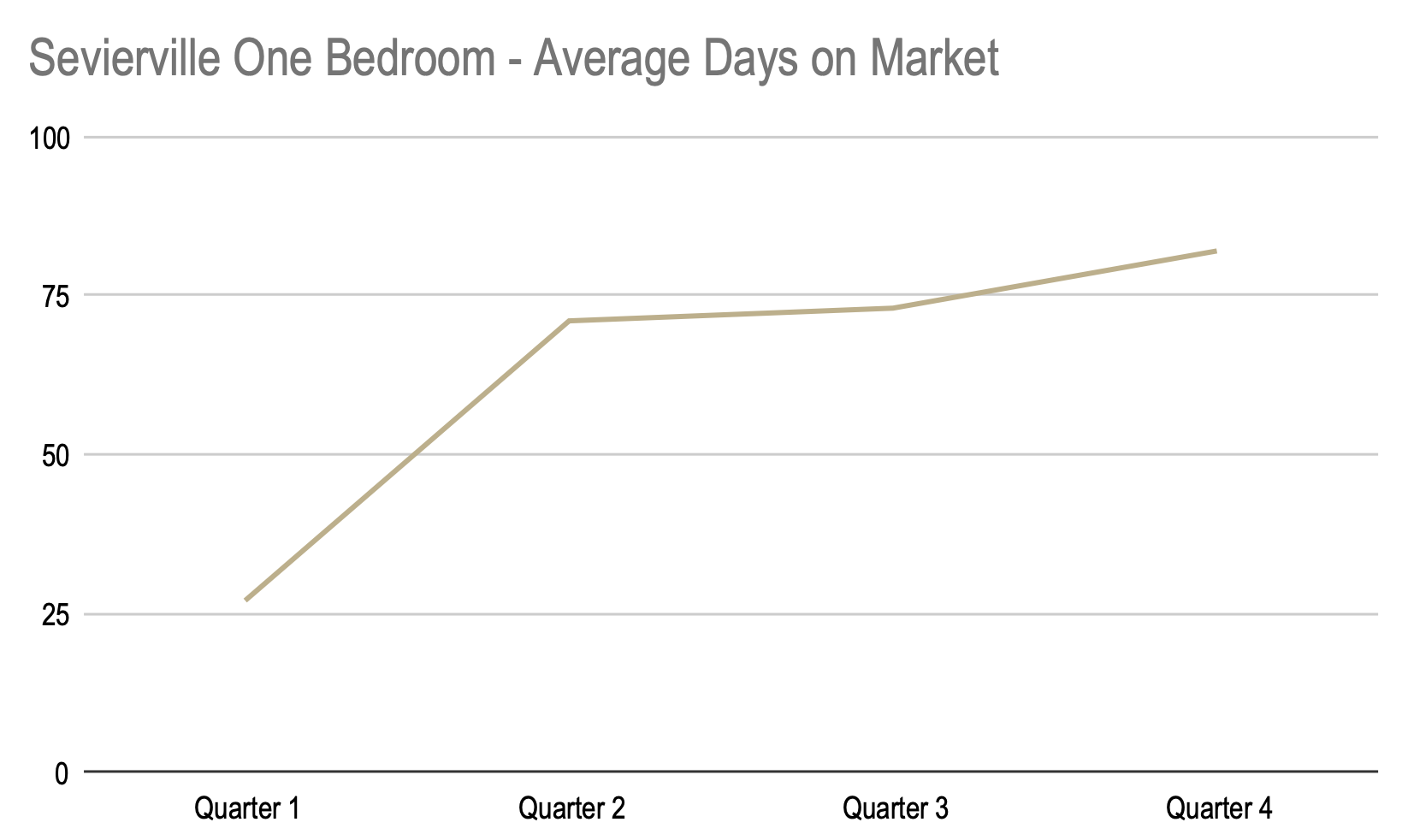We began the year at 27 days on market and ended the year at 82 days on market. For a total increase of 55 days.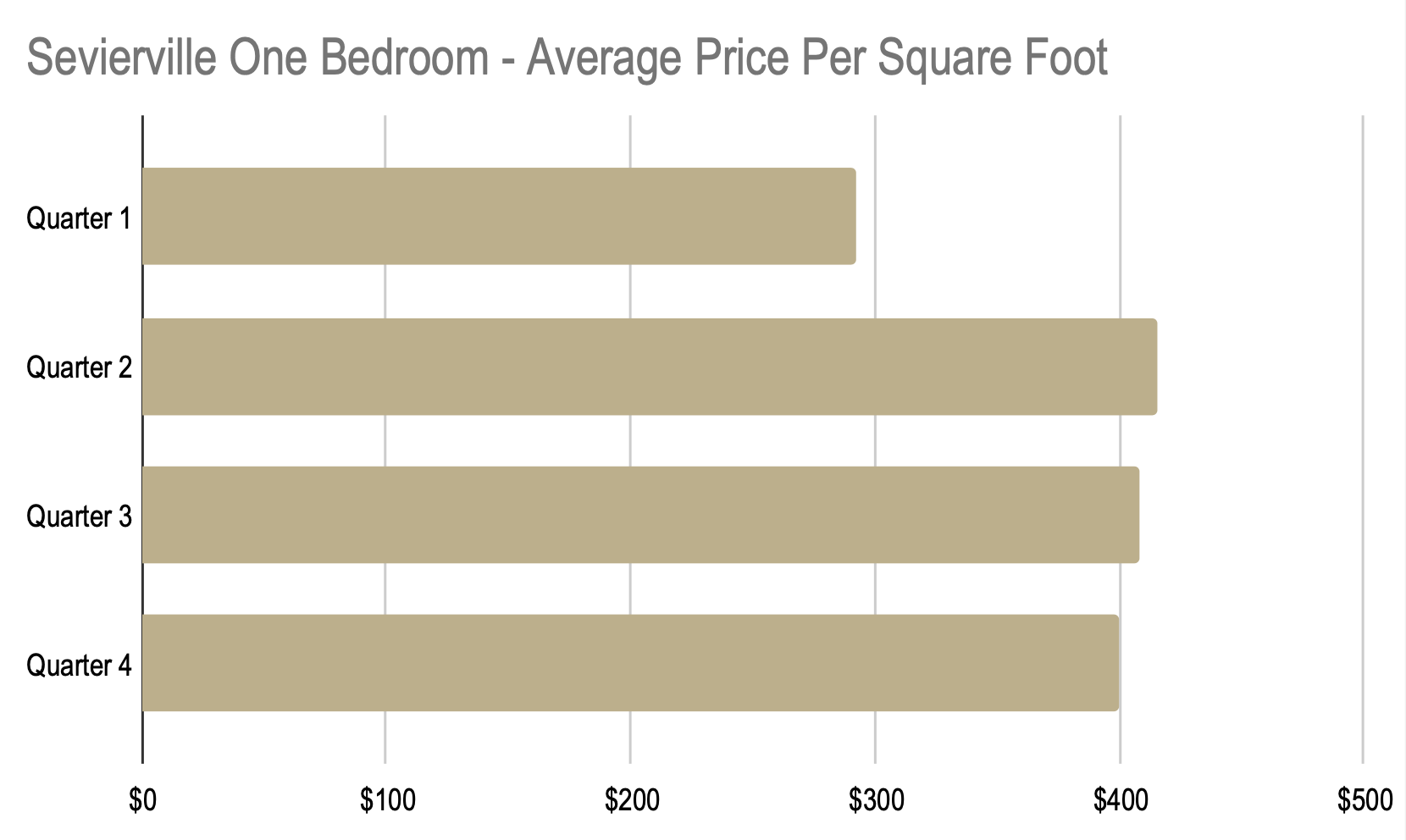### Two Bedroom

We began the year with an average sales price of \$380,764 and ended the year at an average price of \$468,094. For a total increase of \$87,330.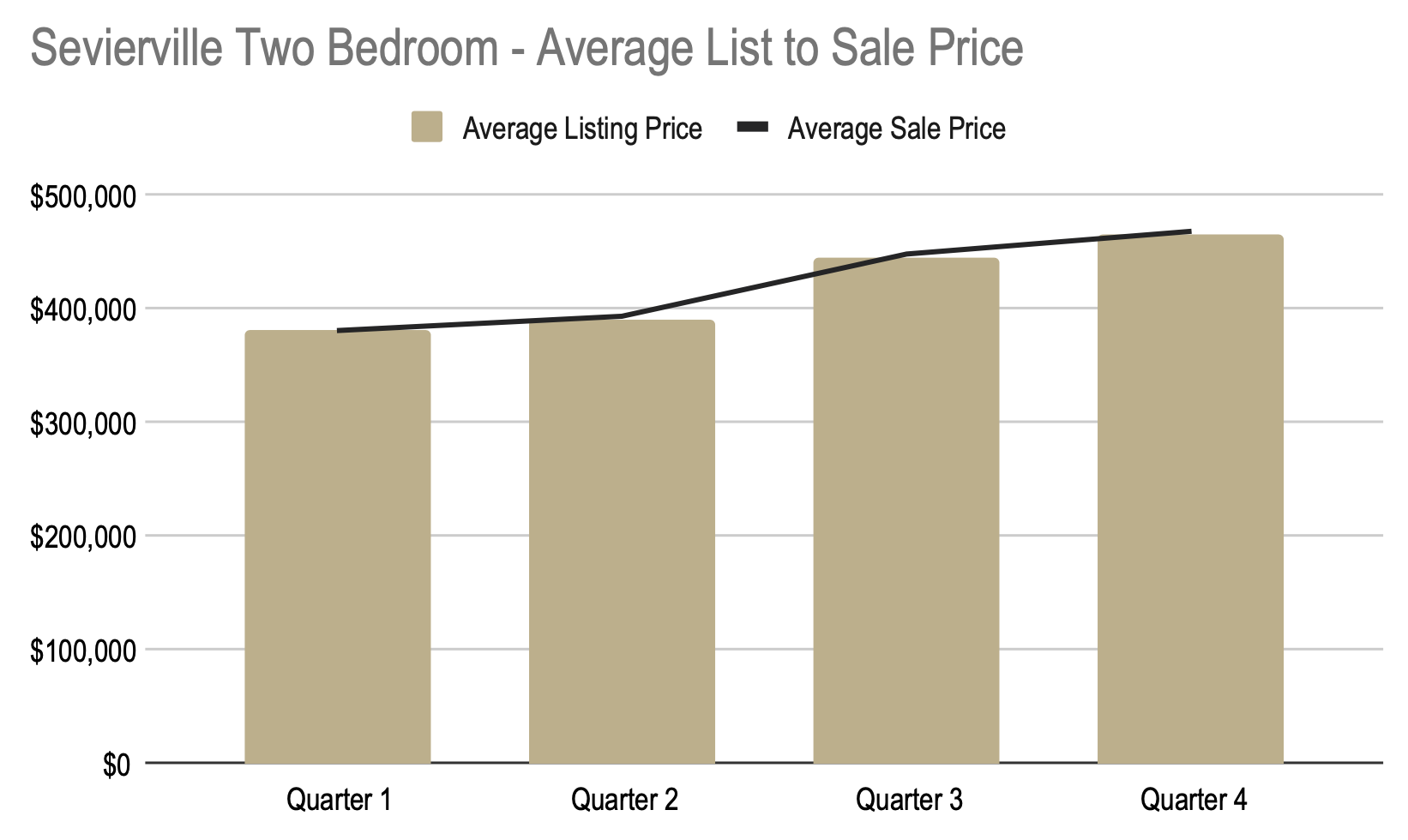We began the year at \$249.84 a square foot and ended the year at \$329.54 a square foot. For a total increase of \$80 per square foot.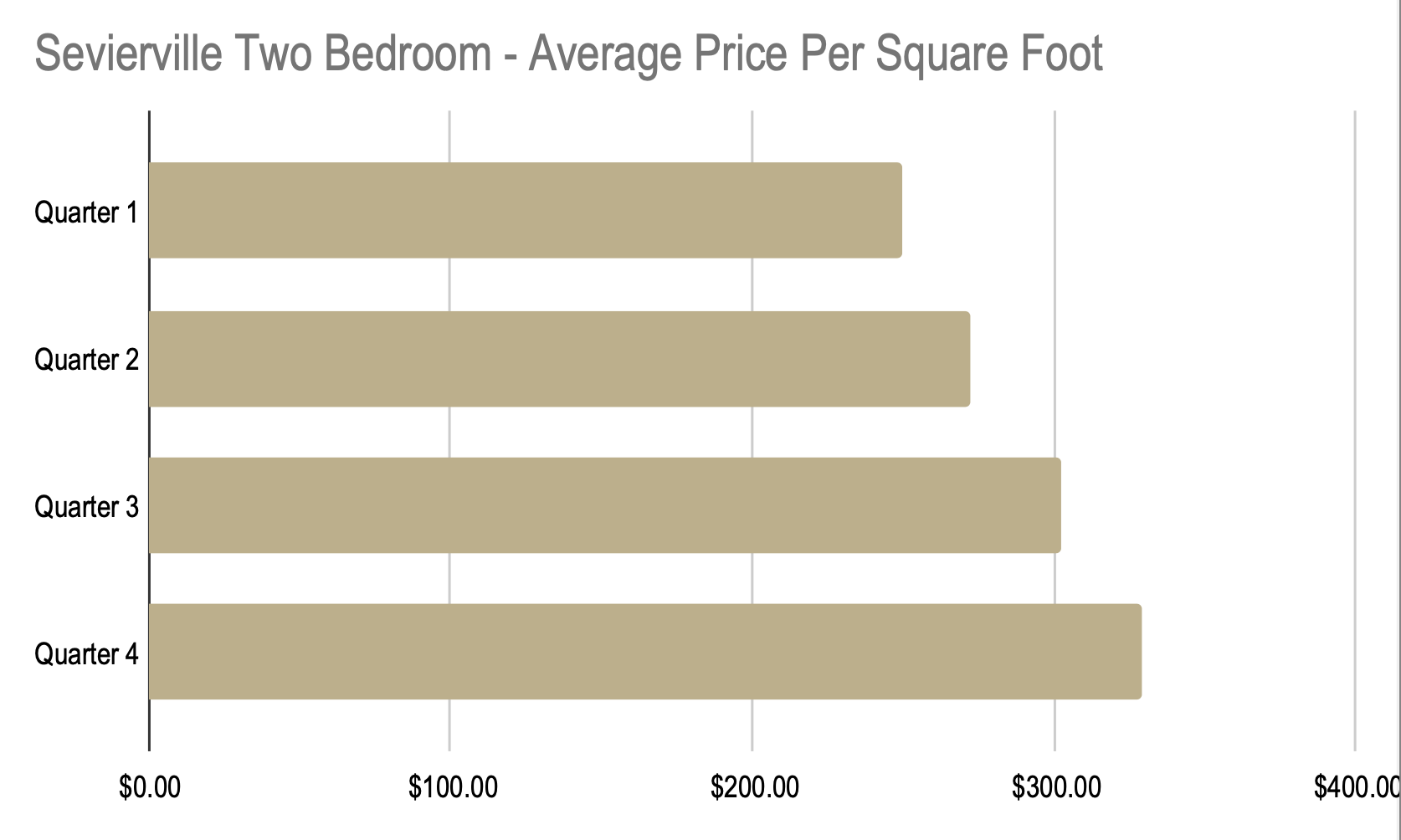We began the year at 69 days on market and ended the year at 81 days on market. For a total increase of 12 days.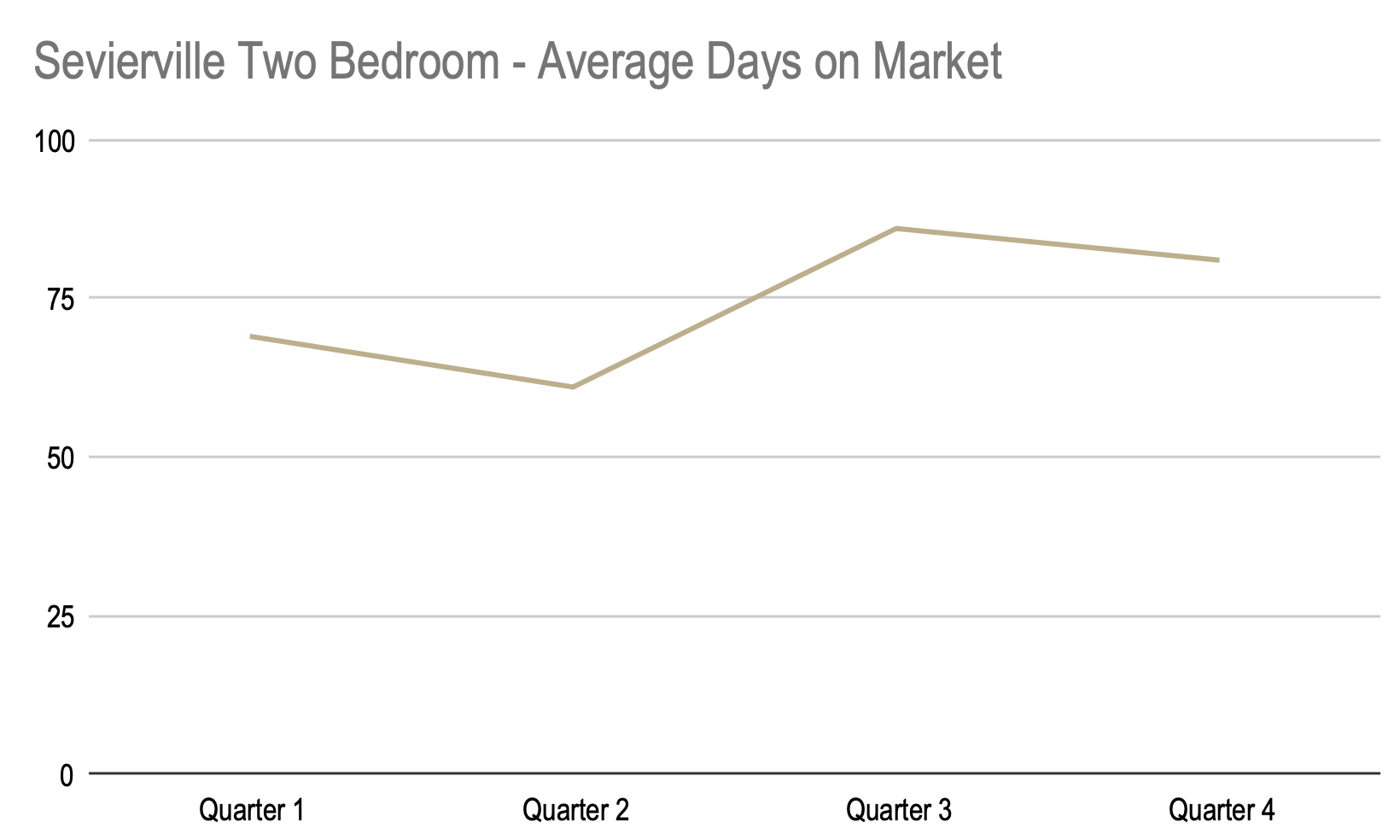### Three Bedroom

We began the year with an average sales price of \$376,368 and ended the year at an average price of \$471,751. For a total increase of \$95,383.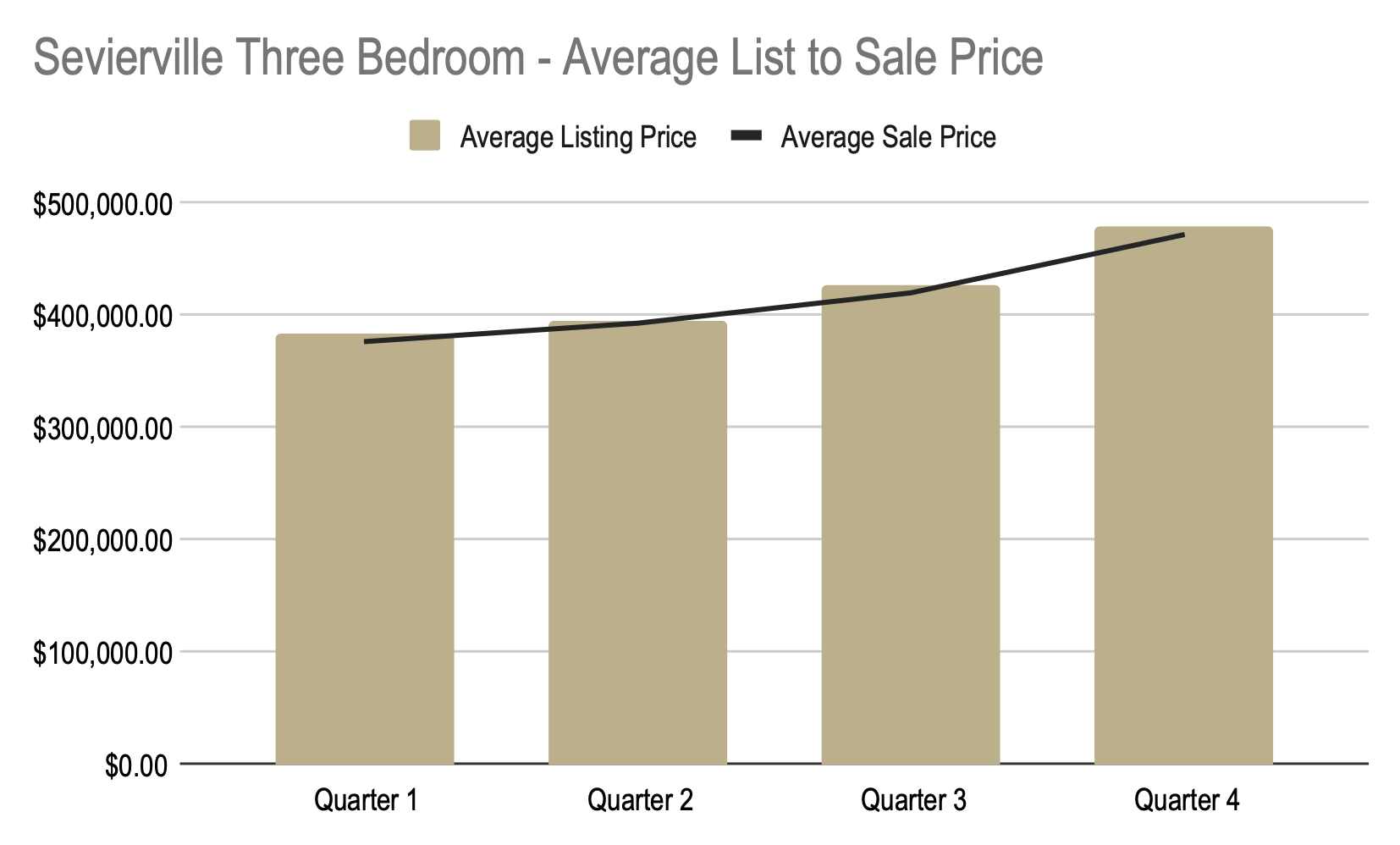We began the year at \$173 a square foot and ended the year at \$223 a square foot. For a total increase of \$50 per square foot.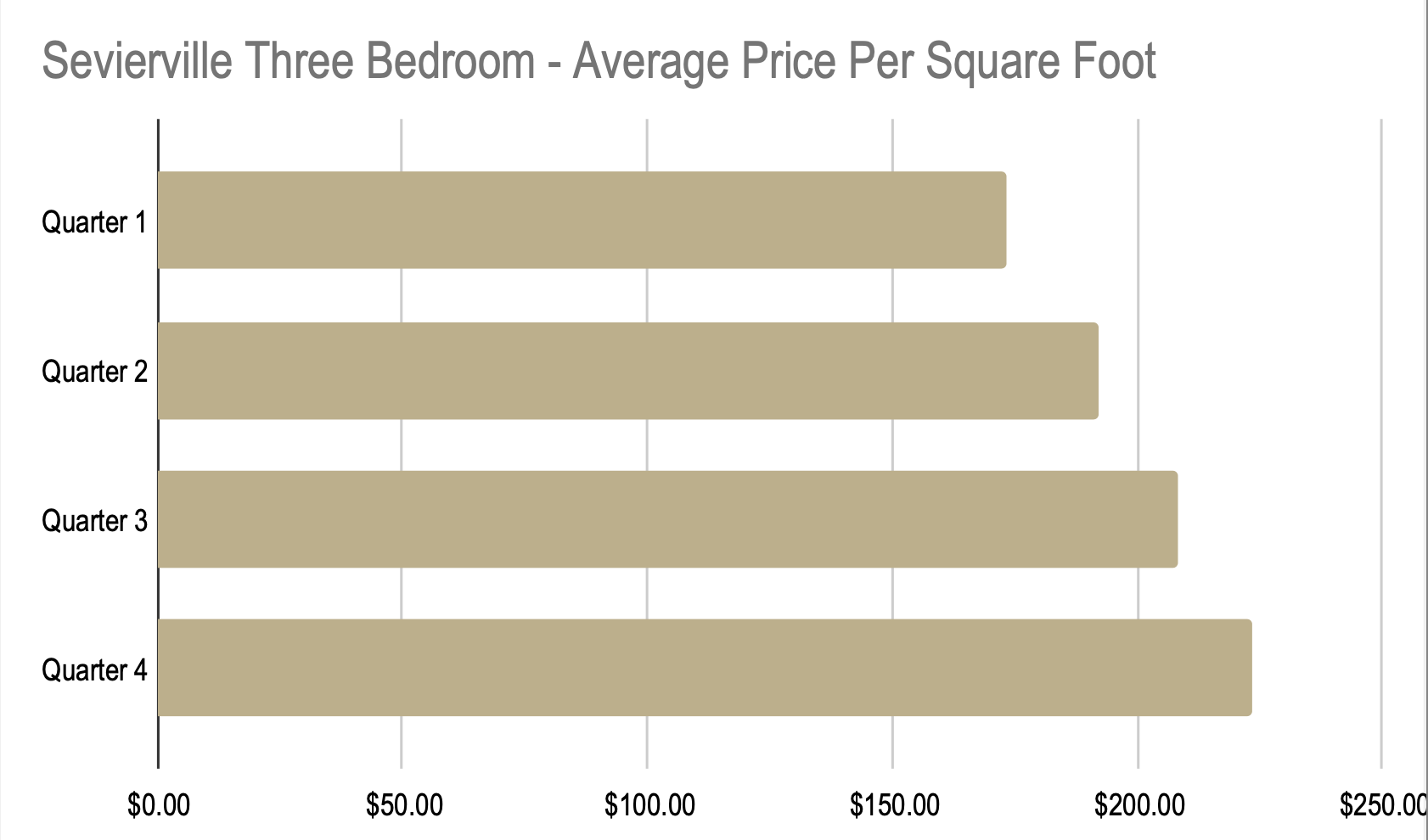We began the year at 81 days on market and ended the year at 87 days on market. For a total increase of 6 days.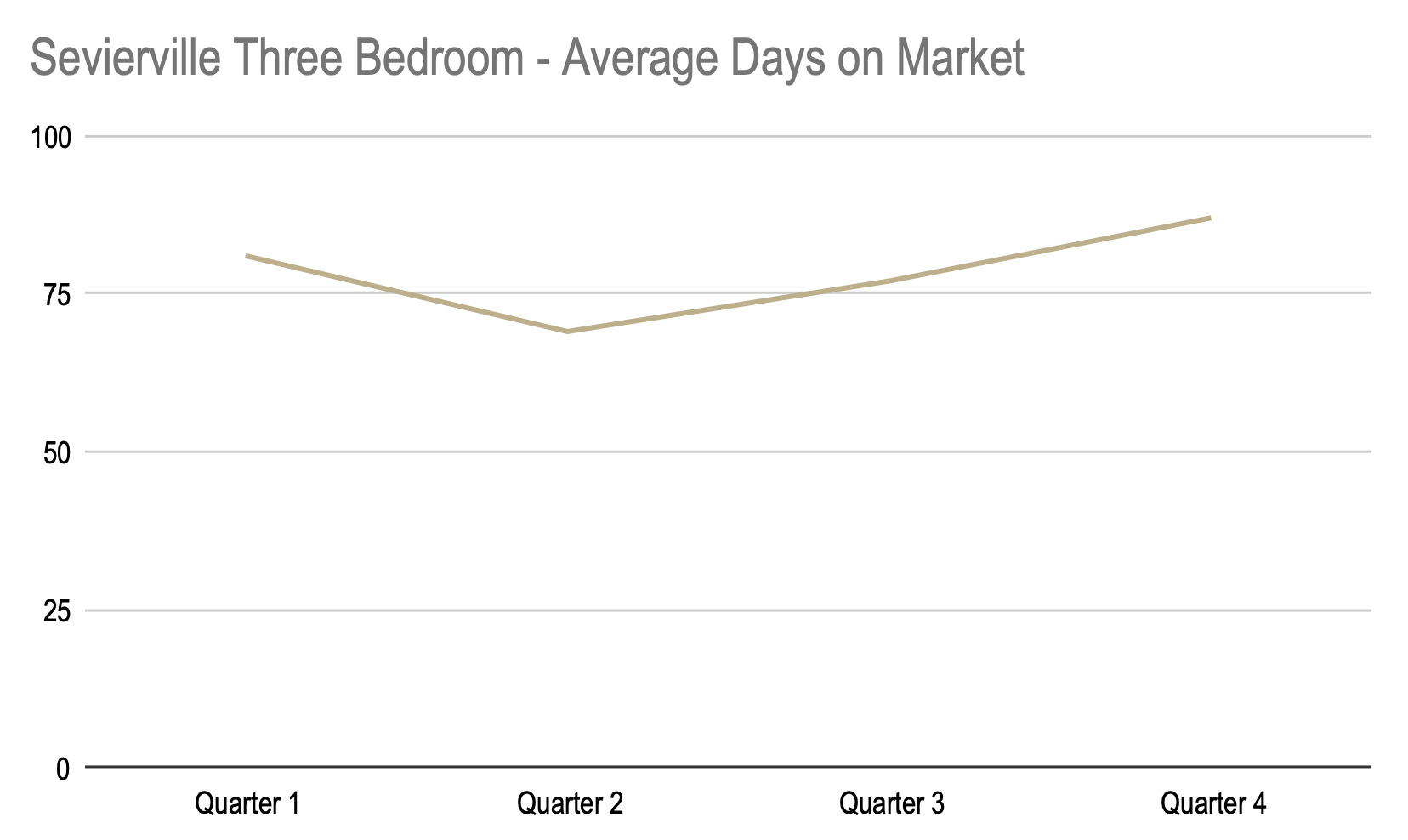### Four Bedroom

We began the year with an average sales price of \$581,324 and ended the year at an average price of\$663,543. For a total increase of \$82,219.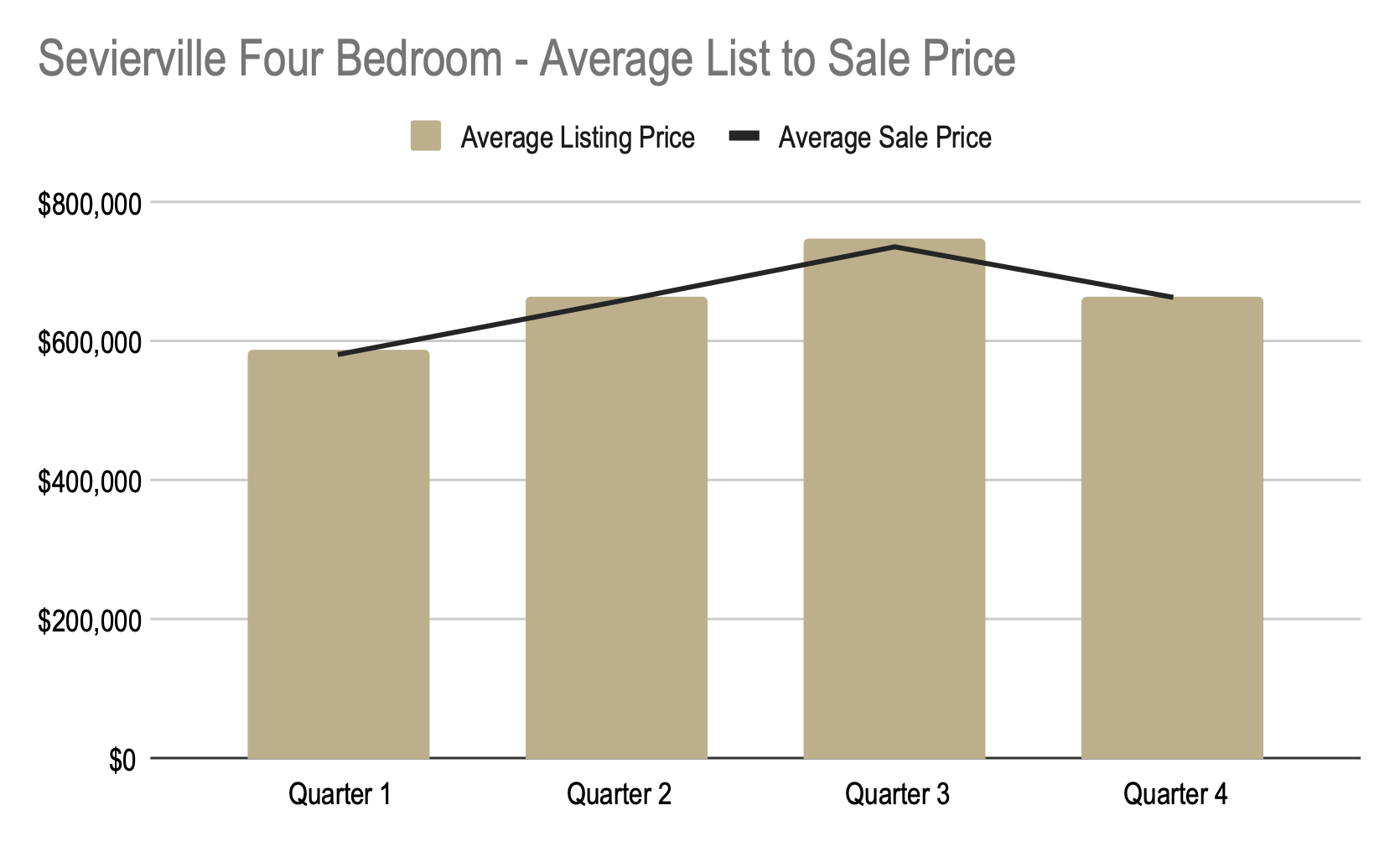We began the year at \$198 a square foot and ended the year at \$236 a square foot. For a total increase of \$38 per square foot.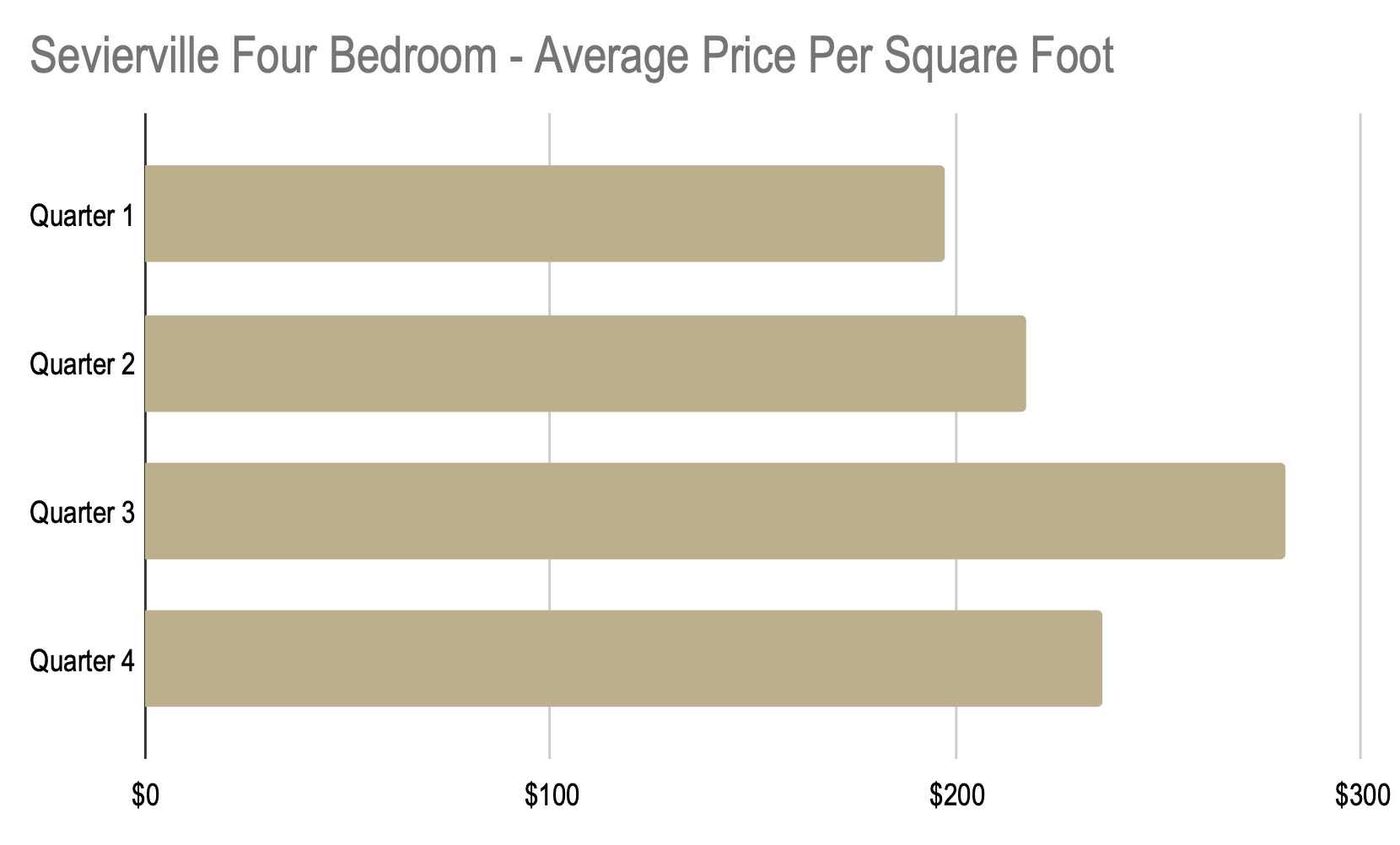We began the year at 134 days on market and ended the year at 90 days on market. For a total decrease of 44 days.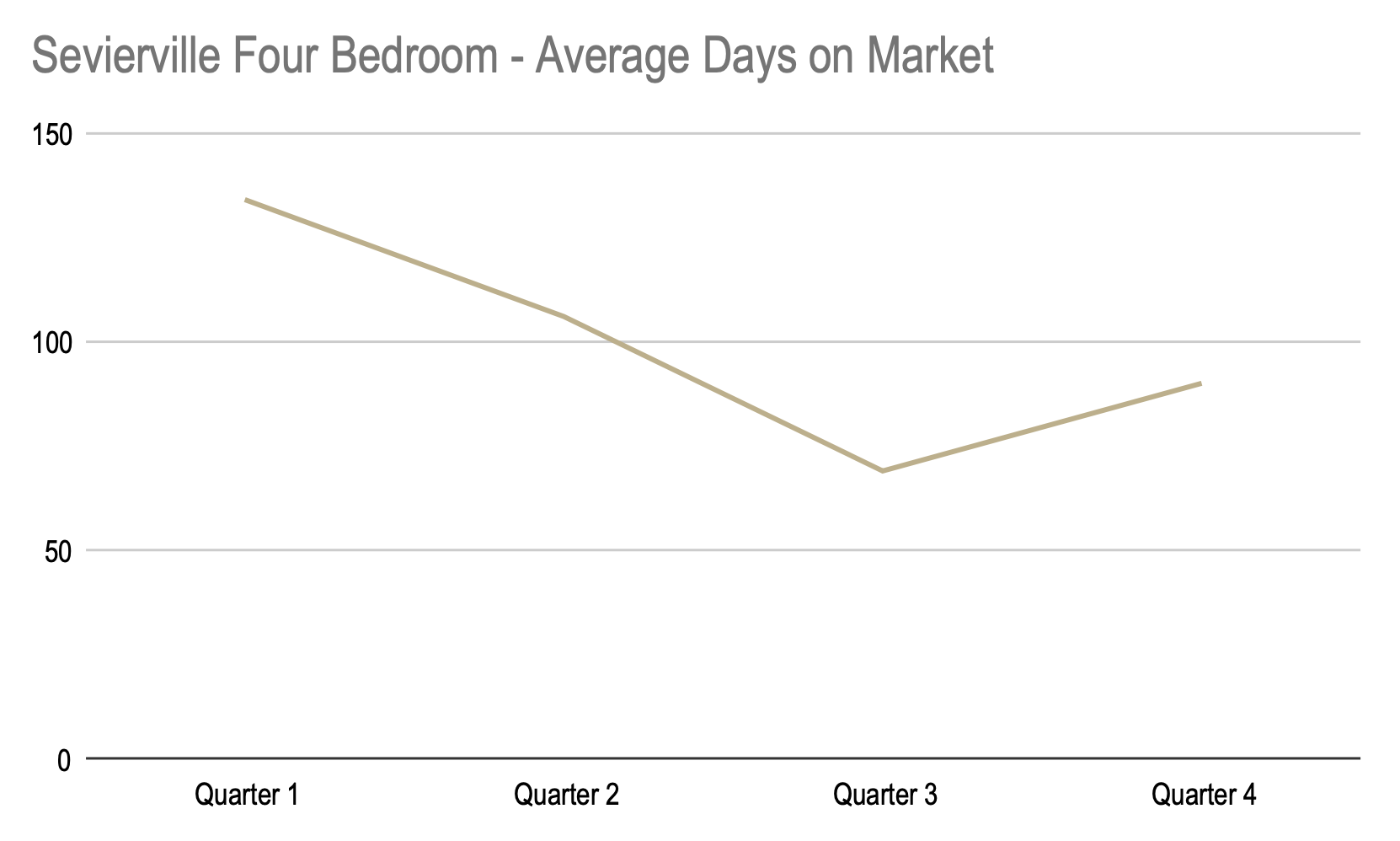### Five+ Bedroom

We began the year with an average sales price of \$1,092,500 and ended the year at an average price of \$1,410,662. For a total increase of \$318,162.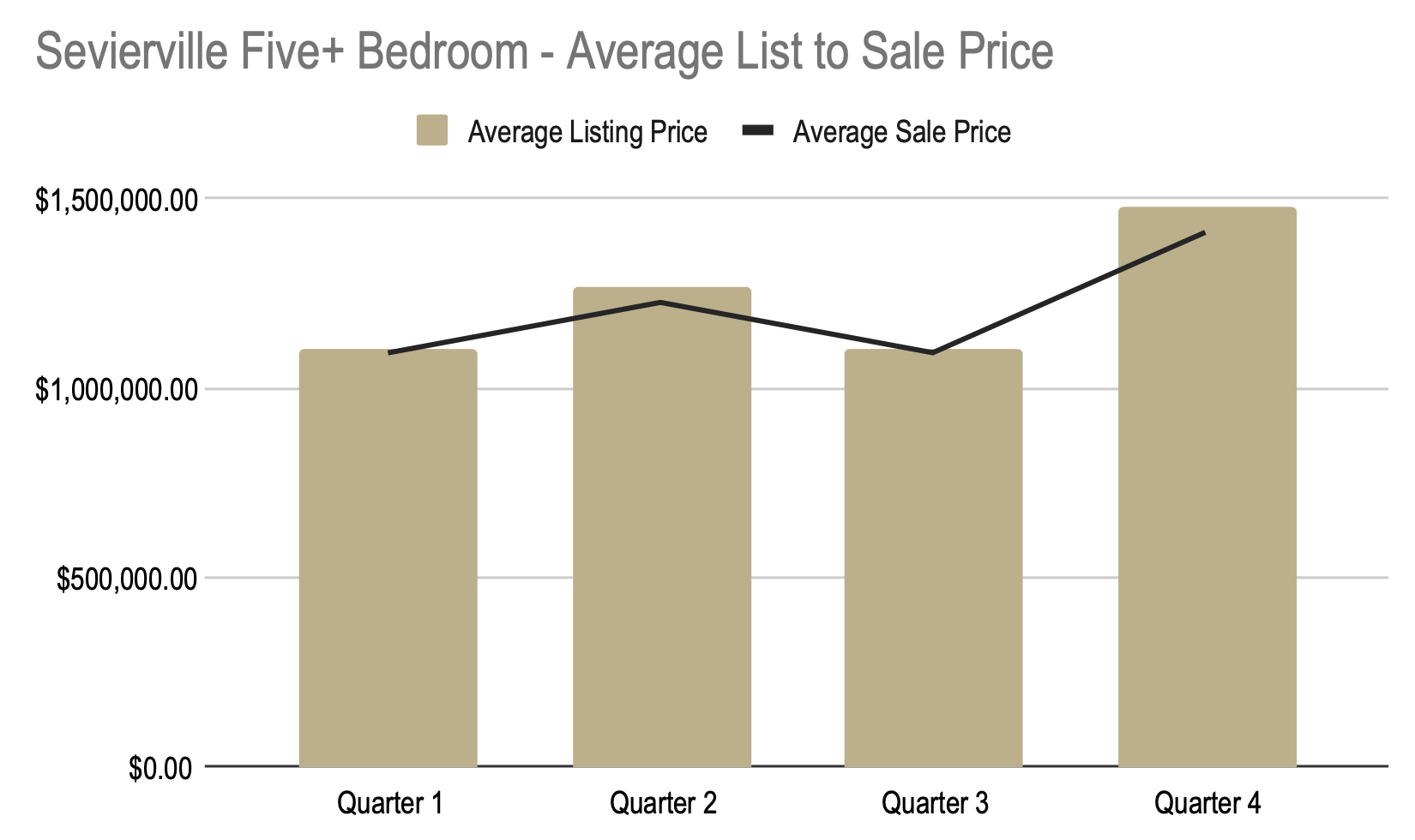We began the year at \$229 a square foot and ended the year at \$305 a square foot. For a total increase of \$76 per square foot.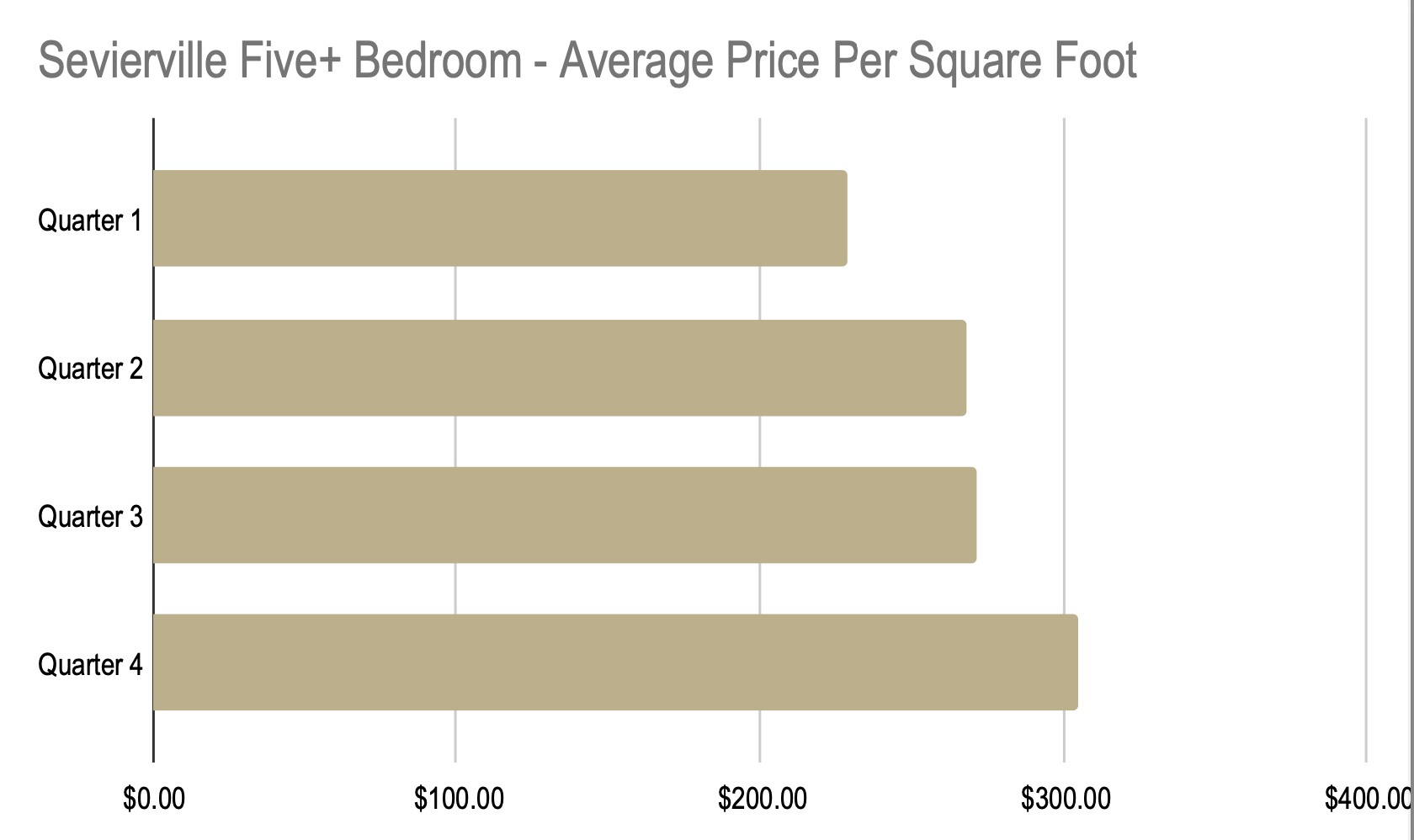We began the year at 112 days on market and ended the year at 151 days on market. We saw a dip during the second and third quarters, but ended the year with an increase of 39 days.